# Chapter 6 Summarized Data Distributions

This chapter explores how to visualize summarized distributions of data.

## 6.1 Making a Basic Histogram

### 6.1.1 Problem

You want to make a histogram.

### 6.1.2 Solution

Use `geom_histogram()` and map a continuous variable to x (Figure 6.1):

``````ggplot(faithful, aes(x = waiting)) +
geom_histogram()``````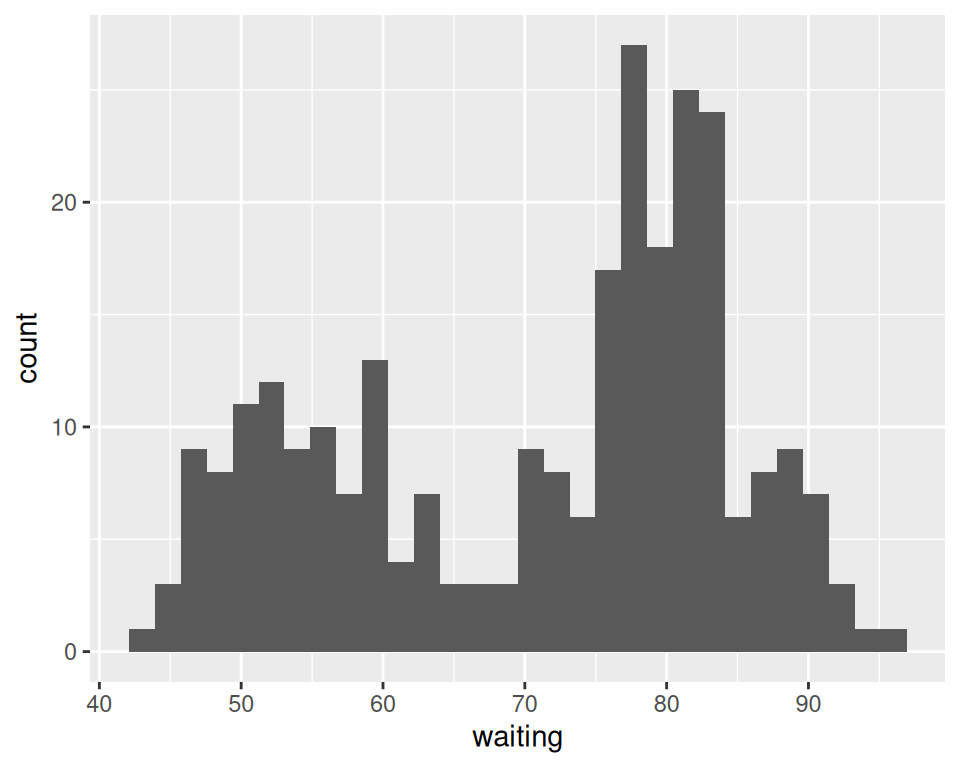Figure 6.1: A basic histogram

### 6.1.3 Discussion

All `geom_histogram()` requires is one column from a data frame or a single vector of data. For this example we’ll use the `faithful` data set, which contains two columns with data about the Old Faithful geyser: `eruptions`, which is the length of each eruption, and `waiting`, which is the length of time to the next eruption. We’ll only use the `waiting` variable in this example:

``````faithful
#>     eruptions waiting
#> 1       3.600      79
#> 2       1.800      54
#> 3       3.333      74
#>  ...<266 more rows>...
#> 270     4.417      90
#> 271     1.817      46
#> 272     4.467      74``````

If you just want to get a quick look at some data that isn’t in a data frame, you can get the same result by passing in `NULL` for the data frame and giving `ggplot()` a vector of values. This would have the same result as the previous code:

``````# Store the values in a simple vector
w <- faithful\$waiting

ggplot(NULL, aes(x = w)) +
geom_histogram()``````

By default, the data is grouped into 30 bins. This number of bins is an arbitrary default value, and may be too fine or too coarse for your data. You can change the size of the bins by specifying the `binwidth`, or you can divide the range of the data into a specific number of bins.

In addition, the default colors – a dark fill without an outline – can make it difficult to see which bar corresponds to which value, so we’ll also change the colors, as shown in Figure 6.2.

``````# Set the width of each bin to 5 (each bin will span 5 x-axis units)
ggplot(faithful, aes(x = waiting)) +
geom_histogram(binwidth = 5, fill = "white", colour = "black")

# Divide the x range into 15 bins
binsize <- diff(range(faithful\$waiting))/15

ggplot(faithful, aes(x = waiting)) +
geom_histogram(binwidth = binsize, fill = "white", colour = "black")``````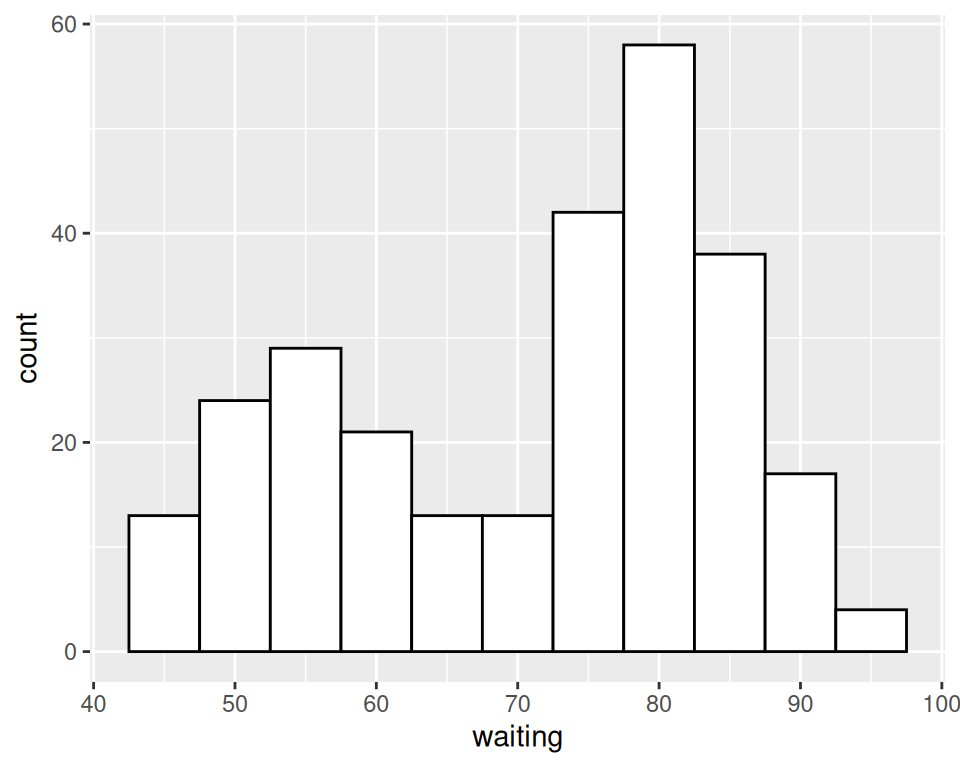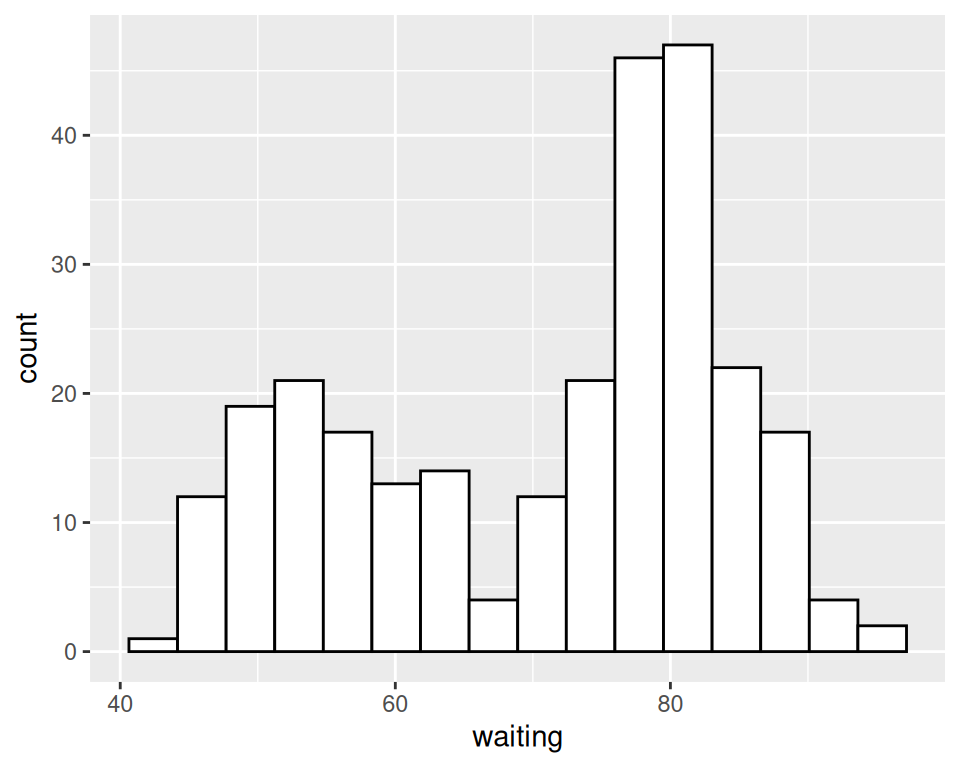Figure 6.2: Histogram with binwidth = 5 and with different colors (left); With 15 bins (right)

Sometimes the appearance of the histogram will be very dependent on the width of the bins and where the boundary points between the bins are. In Figure 6.3, we’ll use a bin width of 8. In the version on the left, we’ll use the origin parameter to put boundaries at 31, 39, 47, etc., while in the version on the right, we’ll shift it over by 4, putting boundaries at 35, 43, 51, etc.:

``````# Save a base plot
faithful_p <- ggplot(faithful, aes(x = waiting))

faithful_p +
geom_histogram(binwidth = 8, fill = "white", colour = "black", boundary = 31)

faithful_p +
geom_histogram(binwidth = 8, fill = "white", colour = "black", boundary = 35)``````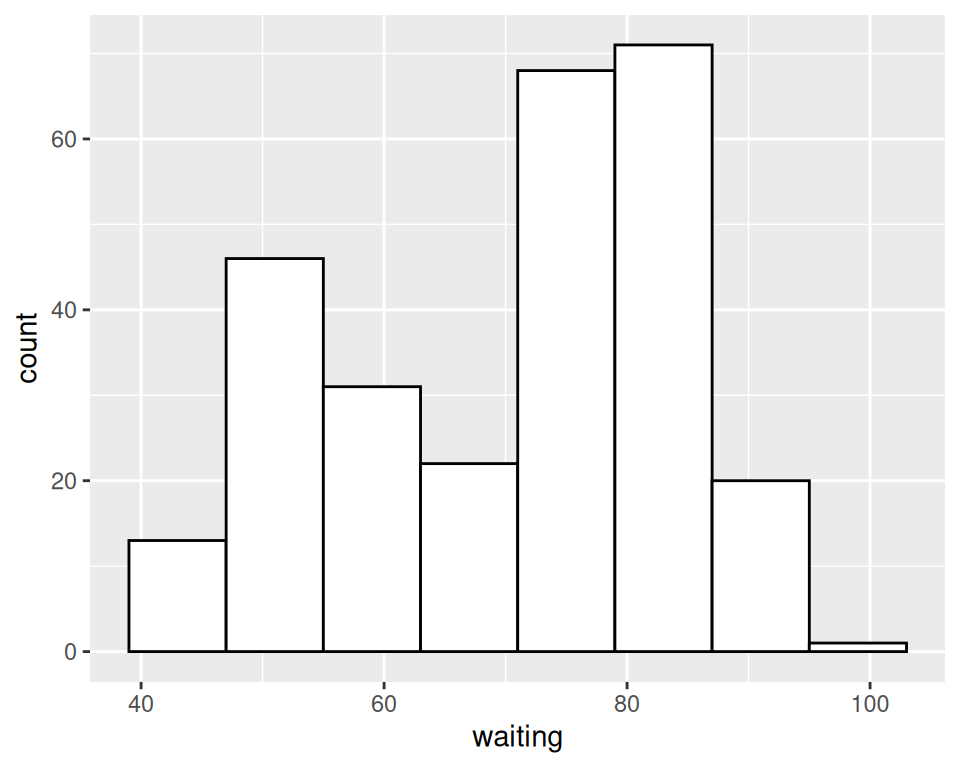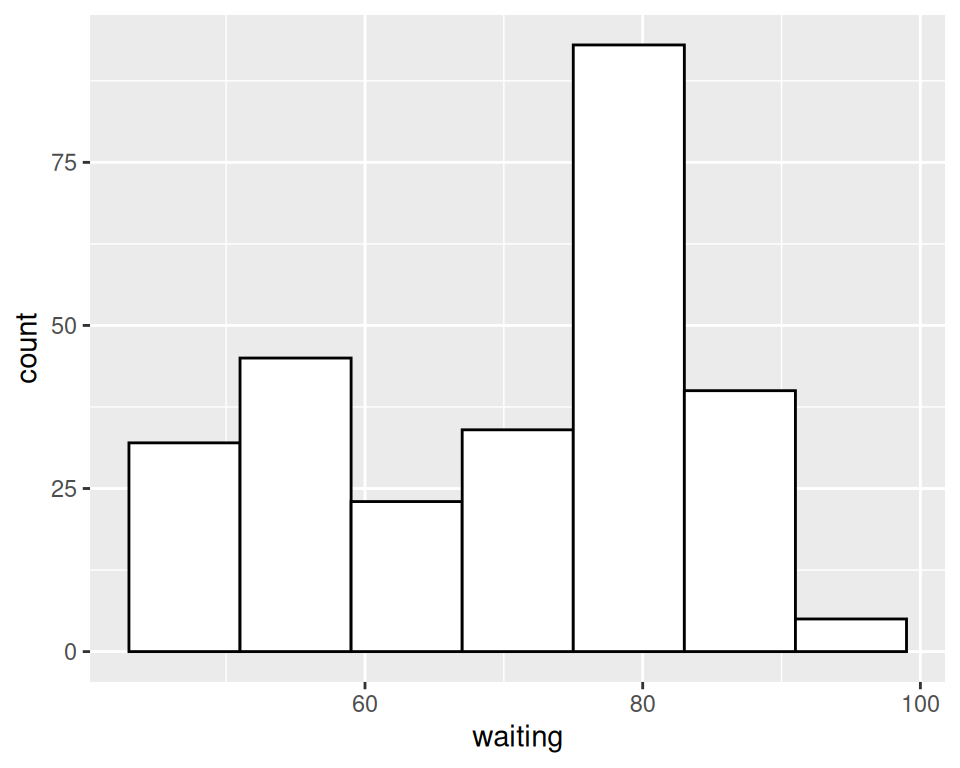Figure 6.3: Different appearance of histograms with the origin at 31 and 35

The results look quite different, even though they have the same bin size. The `faithful` data set is not particularly small, with 272 observations; with smaller data sets, this can be even more of an issue. When visualizing your data, it’s a good idea to experiment with different bin sizes and boundary points.

If your data has discrete values, it may matter that the histogram bins are asymmetrical. They are closed on the lower bound and open on the upper bound. If you have bin boundaries at 1, 2, 3, etc., then the bins will be [1, 2), [2, 3), and so on. In other words, the first bin contains 1 but not 2, and the second bin contains 2 but not 3.

Frequency polygons provide a better way of visualizing multiple distributions without the bars interfering with each other. See Recipe 6.5.

## 6.2 Making Multiple Histograms from Grouped Data

### 6.2.1 Problem

You have grouped data and want to simultaneously make histograms for each data group.

### 6.2.2 Solution

Use `geom_histogram()` and use facets for each group, as shown in Figure 6.4:

``````library(MASS) # Load MASS for the birthwt data set

# Use smoke as the faceting variable
ggplot(birthwt, aes(x = bwt)) +
geom_histogram(fill = "white", colour = "black") +
facet_grid(smoke ~ .)``````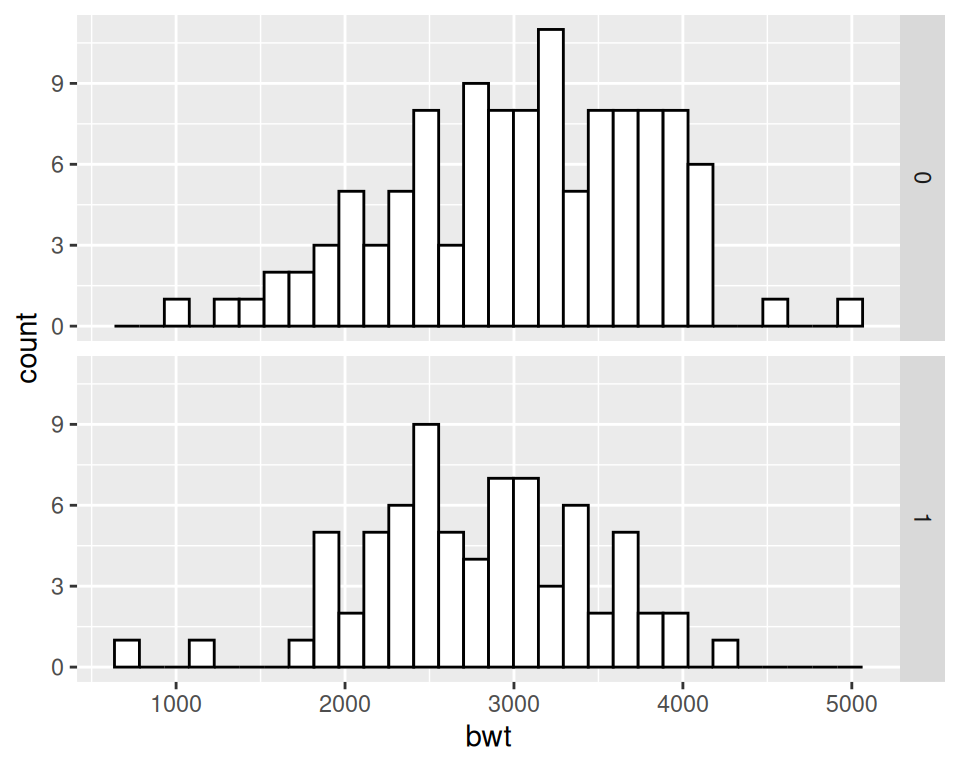Figure 6.4: Two histograms with facets (left); With different facet labels (right)

### 6.2.3 Discussion

To make multiple histograms from grouped data, the data must all be in one data frame, with one column containing a categorical variable used for grouping.

For this example, we used the `birthwt` data set. It contains data about birth weights and a number of risk factors for low birth weight:

``````birthwt
#>    low age lwt race smoke ptl ht ui ftv  bwt
#> 85   0  19 182    2     0   0  0  1   0 2523
#> 86   0  33 155    3     0   0  0  0   3 2551
#> 87   0  20 105    1     1   0  0  0   1 2557
#>  ...<183 more rows>...
#> 82   1  23  94    3     1   0  0  0   0 2495
#> 83   1  17 142    2     0   0  1  0   0 2495
#> 84   1  21 130    1     1   0  1  0   3 2495``````

One problem with the faceted graph is that the facet labels are just 0 and 1, and there’s no label indicating that those values are for whether or not smoking is a risk factor that is present. To change the labels, we change the names of the factor levels. First we’ll take a look at the factor levels, then we’ll assign new factor level names in the same order, and save this new data set as `birthwt_mod`:

``````birthwt_mod <- birthwt
# Convert smoke to a factor and reassign new names
birthwt_mod\$smoke <- recode_factor(birthwt_mod\$smoke, '0' = 'No Smoke', '1' = 'Smoke')``````

Now when we plot our modified data frame, our desired labels appear (Figure 6.5).

``````ggplot(birthwt_mod, aes(x = bwt)) +
geom_histogram(fill = "white", colour = "black") +
facet_grid(smoke ~ .)``````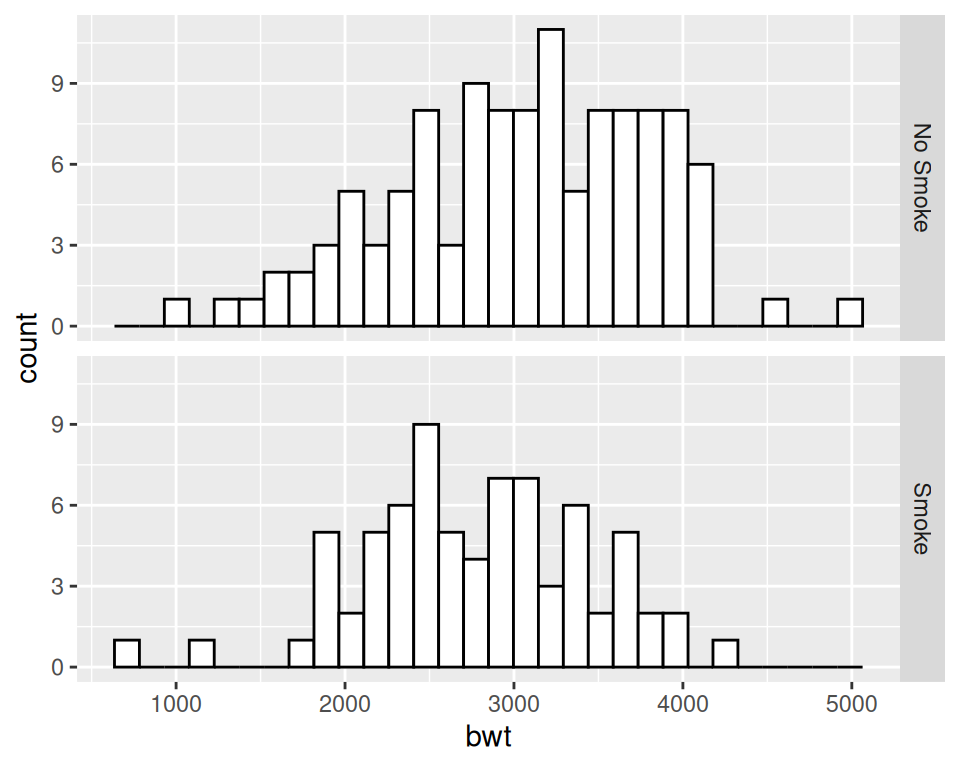Figure 6.5: Histograms with new facet labels

With facets, the axes have the same y scaling in each facet. If your groups have different sizes, it might be hard to compare the shapes of the distributions of each one. For example, see what happens when we facet the birth weights by `race` (Figure 6.6, left):

``````ggplot(birthwt, aes(x = bwt)) +
geom_histogram(fill = "white", colour = "black") +
facet_grid(race ~ .)``````

To allow the y scales to be resized independently (Figure 6.6, right), use `scales = "free"`. Note that this will only allow the y scales to be free – the x scales will still be fixed because the histograms are aligned with respect to that axis:

``````ggplot(birthwt, aes(x = bwt)) +
geom_histogram(fill = "white", colour = "black") +
facet_grid(race ~ ., scales = "free")``````Figure 6.6: Histograms with the default fixed scales (left); With scales = “free” (right)

Another approach is to map the grouping variable to `fill`, as shown in Figure 6.7. The grouping variable must be a factor or a character vector. In the `birthwt` data set, the desired grouping variable, `smoke`, is stored as a number, so we’ll use the `birthwt_mod` data set we created above, in which smoke is a factor:

``````# Map smoke to fill, make the bars NOT stacked, and make them semitransparent
ggplot(birthwt_mod, aes(x = bwt, fill = smoke)) +
geom_histogram(position = "identity", alpha = 0.4)``````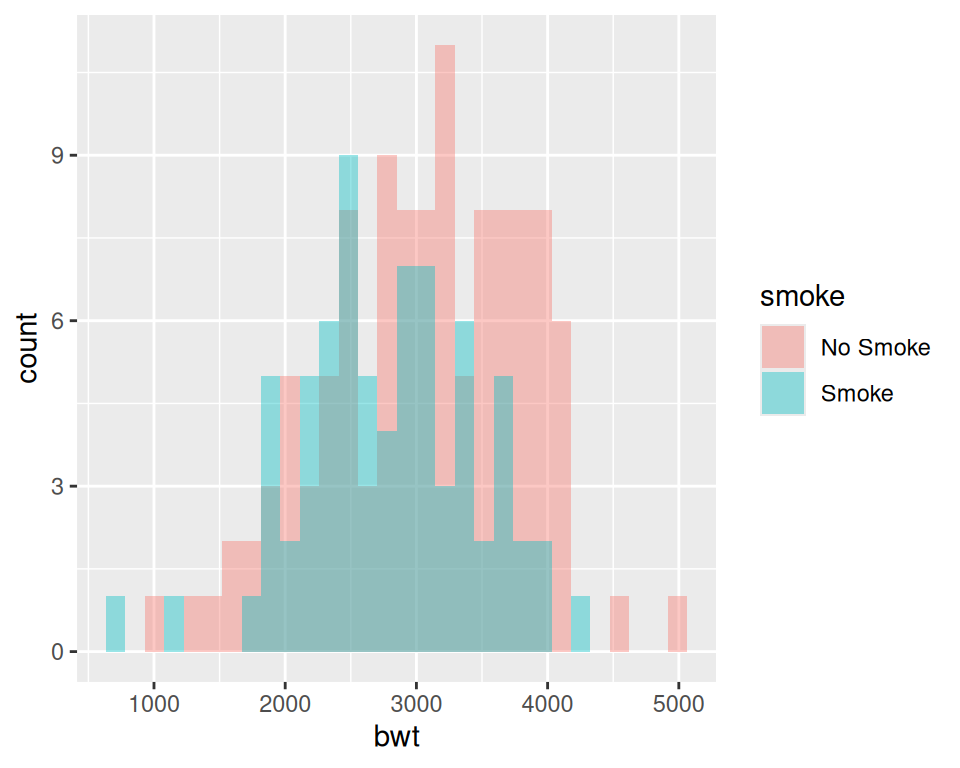Figure 6.7: Multiple histograms with different fill colors

Specifying `position = "identity"` is important. Without it, ggplot will stack the histogram bars on top of each other vertically, making it much more difficult to see the distribution of each group.

## 6.3 Making a Density Curve

### 6.3.1 Problem

You want to make a kernel density estimate curve.

### 6.3.2 Solution

Use `geom_density()` and map a continuous variable to x (Figure 6.8):

``````ggplot(faithful, aes(x = waiting)) +
geom_density()``````

If you don’t like the lines along the side and bottom, you can use `geom_line(stat = "density")` (see Figure 6.8, right):

``````# expand_limits() increases the y range to include the value 0
ggplot(faithful, aes(x = waiting)) +
geom_line(stat = "density") +
expand_limits(y = 0)``````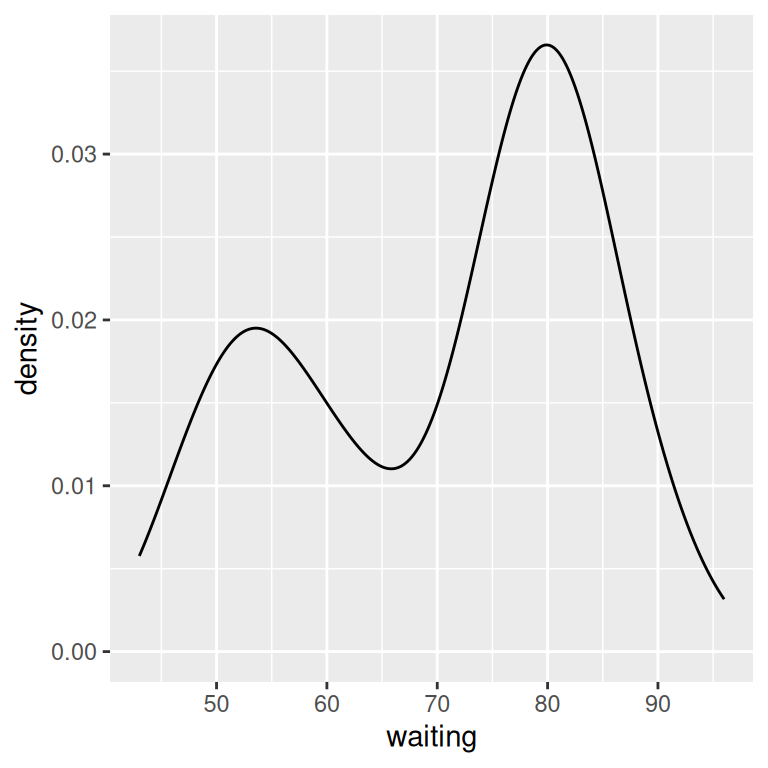Figure 6.8: A kernel density estimate curve with `geom_density()` (left); With `geom_line()` (right)

### 6.3.3 Discussion

Like `geom_histogram()`, `geom_density()` requires just one column from a data frame. For this example, we’ll use the `faithful` data set, which contains two columns of data about the Old Faithful geyser: `eruptions`, which is the length of each eruption, and `waiting`, which is the length of time until the next eruption. We’ll only use the `waiting` column in this example:

``````faithful
#>     eruptions waiting
#> 1       3.600      79
#> 2       1.800      54
#> 3       3.333      74
#>  ...<266 more rows>...
#> 270     4.417      90
#> 271     1.817      46
#> 272     4.467      74``````

The second method of using `geom_line(stat = "density")` tells `geom_line()` to use the “density” statistical transformation. This is essentially the same as the first method, using `geom_density()`, except the former draws it with a closed polygon.

As with `geom_histogram()`, if you just want to get a quick look at data that isn’t in a data frame, you can get the same result by passing in `NULL` for the data and giving ggplot a vector of values. This would have the same result as the first solution:

``````# Store the values in a simple vector
w <- faithful\$waiting

ggplot(NULL, aes(x = w)) +
geom_density()``````

A kernel density curve is an estimate of the population distribution, based on the sample data. The amount of smoothing depends on the kernel bandwidth: the larger the bandwidth, the more smoothing there is. The bandwidth can be set with the `adjust` parameter, which has a default value of 1. Figure 6.9 shows what happens with a smaller and larger value of `adjust`:

``````ggplot(faithful, aes(x = waiting)) +
geom_line(stat = "density") +
geom_line(stat = "density", adjust = .25, colour = "red") +
geom_line(stat = "density", adjust = 2, colour = "blue")``````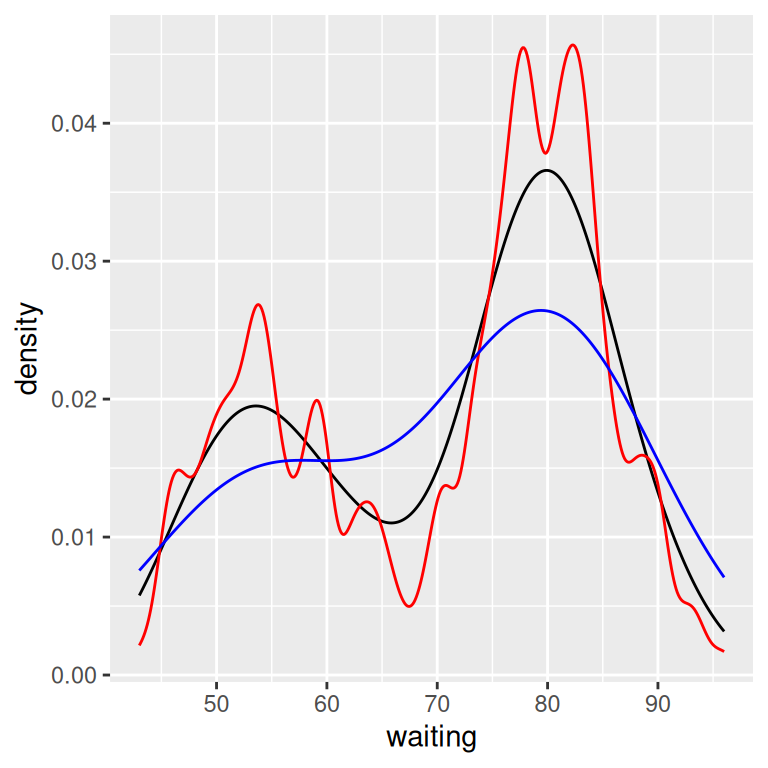Figure 6.9: Density curves with adjust set to .25 (red), default value of 1 (black), and 2 (blue)

In this example, the x range is automatically set so that it contains the data, but this results in the edge of the curve getting clipped. To show more of the curve, set the x limits (Figure 6.10). We’ll also add an 80% transparent fill, with `alpha = .2`:

``````ggplot(faithful, aes(x = waiting)) +
geom_density(fill = "blue", alpha = .2) +
xlim(35, 105)

# This draws a blue polygon with geom_density(), then adds a line on top
ggplot(faithful, aes(x = waiting)) +
geom_density(fill = "blue", alpha = .2, colour = NA) +
xlim(35, 105) +
geom_line(stat = "density")``````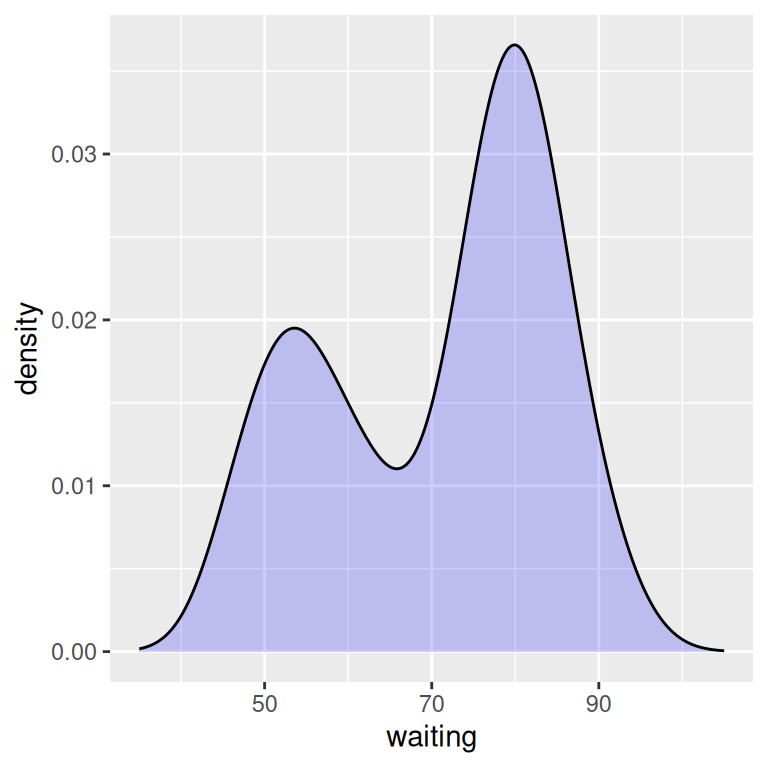Figure 6.10: Density curve with wider x limits and a semitransparent fill (left); In two parts, with `geom_density()` and `geom_line()` (right)

If this edge-clipping happens with your data, it might mean that your curve is too smooth. If the curve is much wider than your data, it might not be the best model of your data, or it could be because you have a small data set.

To compare the theoretical and observed distributions of your data, you can overlay the density curve with the histogram. Since the y values for the density curve are small (the area under the curve always sums to 1), it would be barely visible if you overlaid it on a histogram without any transformation. To solve this problem, you can scale down the histogram to match the density curve with the mapping `y = ..density..`. Here we’ll add `geom_histogram()` first, and then layer `geom_density()` on top (Figure 6.11):

``````ggplot(faithful, aes(x = waiting, y = ..density..)) +
geom_histogram(fill = "cornsilk", colour = "grey60", size = .2) +
geom_density() +
xlim(35, 105)
#> Warning: Removed 1 rows containing missing values (geom_bar).``````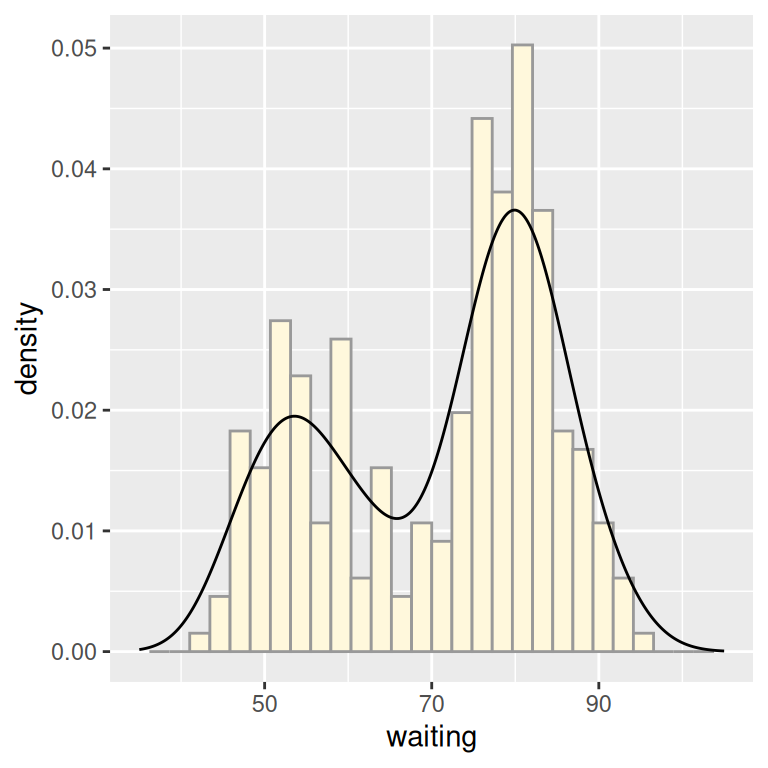Figure 6.11: Density curve overlaid on a histogram

See Recipe 6.9 for information on violin plots, which are another way of representing density curves and may be more appropriate for comparing multiple distributions.

## 6.4 Making Multiple Density Curves from Grouped Data

### 6.4.1 Problem

You want to make density curves of multiple groups of data.

### 6.4.2 Solution

Use `geom_density()`, and map the grouping variable to an aesthetic like `colour` or `fill`, as shown in Figure 6.12. The grouping variable must be a factor or a character vector. In the `birthwt` data set, the desired grouping variable, `smoke`, is stored as a number, so we have to convert it to a factor first.

``````library(MASS) # Load MASS for the birthwt data set

birthwt_mod <- birthwt %>%
mutate(smoke = as.factor(smoke)) # Convert smoke to a factor

# Map smoke to colour
ggplot(birthwt_mod, aes(x = bwt, colour = smoke)) +
geom_density()

# Map smoke to fill and make the fill semitransparent by setting alpha
ggplot(birthwt_mod, aes(x = bwt, fill = smoke)) +
geom_density(alpha = .3)``````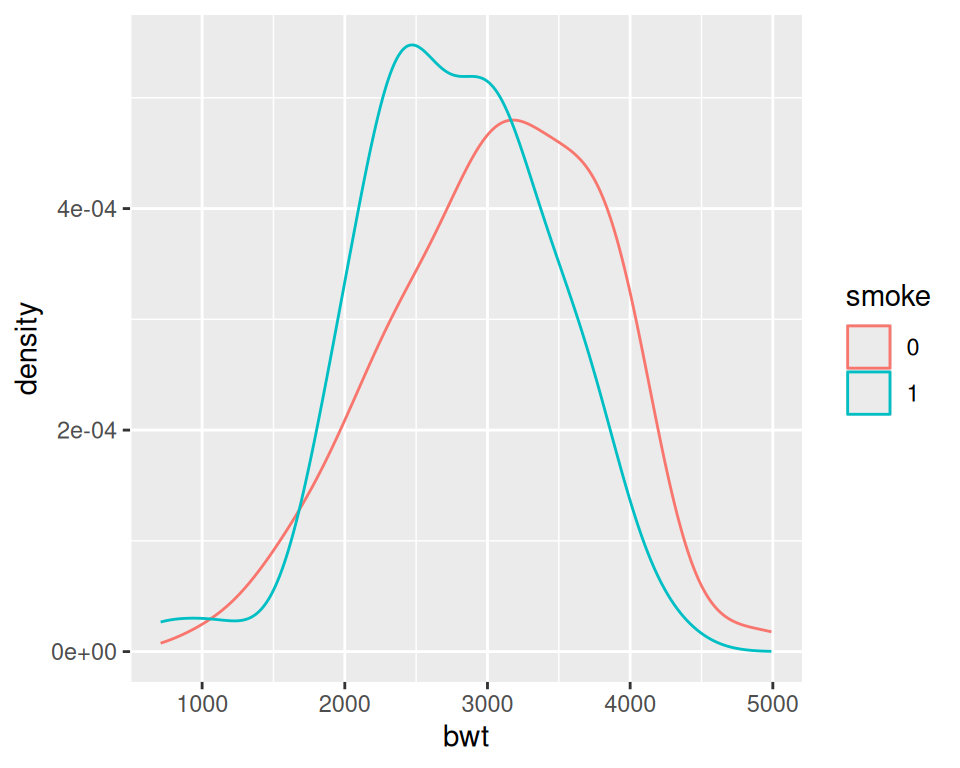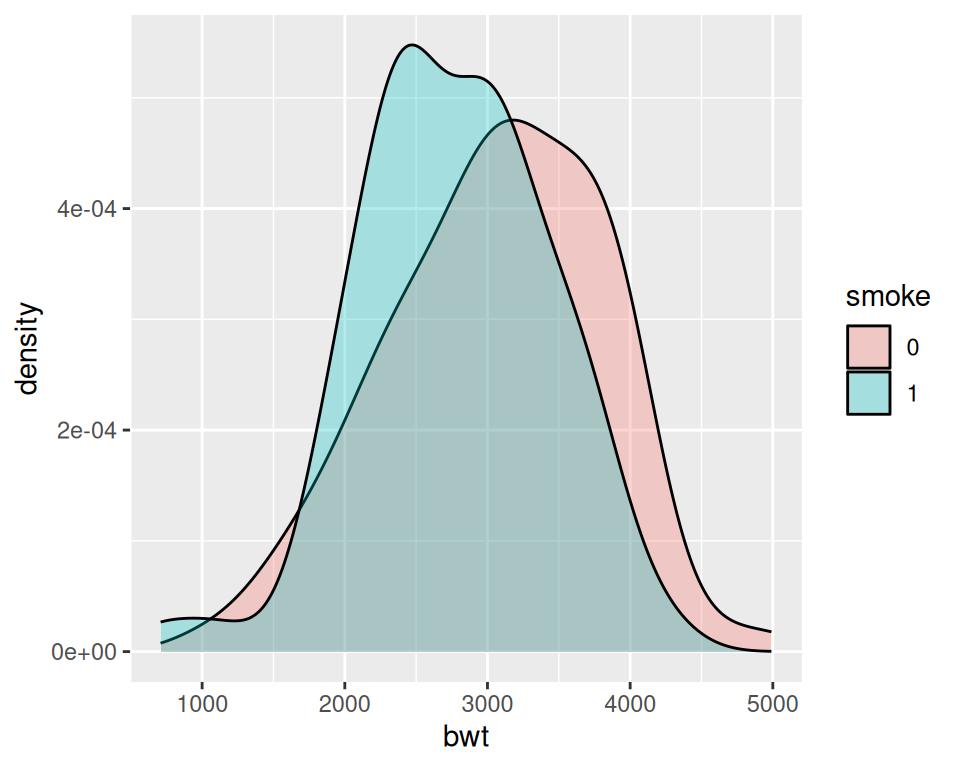Figure 6.12: Different line colors for each group (left); Different semitransparent fill colors for each group (right)

### 6.4.3 Discussion

To make these plots, the data must all be in one data frame, with one column containing a categorical variable used for grouping.

For this example, we used the `birthwt` data set. It contains data about birth weights and a number of risk factors for low birth weight:

``````birthwt
#>    low age lwt race smoke ptl ht ui ftv  bwt
#> 85   0  19 182    2     0   0  0  1   0 2523
#> 86   0  33 155    3     0   0  0  0   3 2551
#> 87   0  20 105    1     1   0  0  0   1 2557
#>  ...<183 more rows>...
#> 82   1  23  94    3     1   0  0  0   0 2495
#> 83   1  17 142    2     0   0  1  0   0 2495
#> 84   1  21 130    1     1   0  1  0   3 2495``````

We looked at the relationship between `smoke` (smoking) and `bwt` (birth weight in grams). The value of `smoke` is either 0 or 1, but since it’s stored as a numeric vector, ggplot doesn’t know that it should be treated as a categorical variable. To make it so ggplot knows to treat `smoke` as categorical, we can either convert that column of the data frame to a factor, or tell ggplot to treat it as a factor by using `factor(smoke)` inside of the `aes()` statement. For these examples, we converted `smoke` to a factor.

Another method for visualizing the distributions is to use facets, as shown in Figure 6.13. We can align the facets vertically or horizontally. Here we’ll align them vertically so that it’s easy to compare the two distributions:

``````ggplot(birthwt_mod, aes(x = bwt)) +
geom_density() +
facet_grid(smoke ~ .)``````

One problem with the faceted graph is that the facet labels are just 0 and 1, and there’s no label indicating that those values are for smoke. To change the labels, we need to change the names of the factor levels. First we’ll take a look at the factor levels, then we’ll assign new factor level names:

``````levels(birthwt_mod\$smoke)
#>  "0" "1"

birthwt_mod\$smoke <- recode(birthwt_mod\$smoke, '0' = 'No Smoke', '1' = 'Smoke')``````

Now when we plot our modified data frame, our desired labels appear (Figure 6.13, right):

``````ggplot(birthwt_mod, aes(x = bwt)) +
geom_density() +
facet_grid(smoke ~ .)``````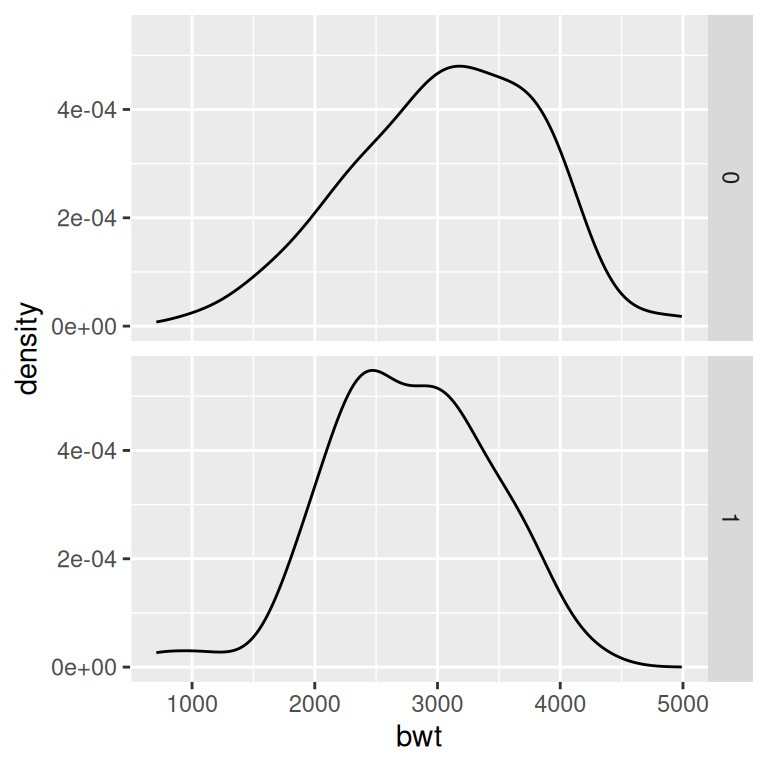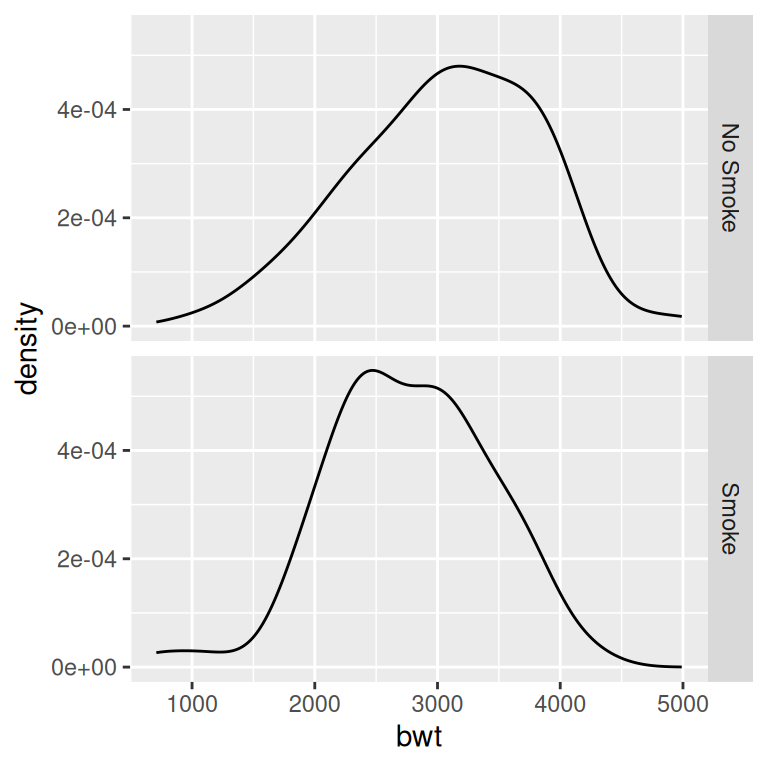Figure 6.13: Density curves with facets (left); With different facet labels (right)

If you want to see the histograms along with the density curves, the best option is to use facets, since other methods of visualizing both histograms in a single graph can be difficult to interpret. To do this, map `y = ..density..`, so that the histogram is scaled down to the height of the density curves. In this example, we’ll also make the histogram bars a little less prominent by changing the colors (Figure 6.14):

``````ggplot(birthwt_mod, aes(x = bwt, y = ..density..)) +
geom_histogram(binwidth = 200, fill = "cornsilk", colour = "grey60", size = .2) +
geom_density() +
facet_grid(smoke ~ .)``````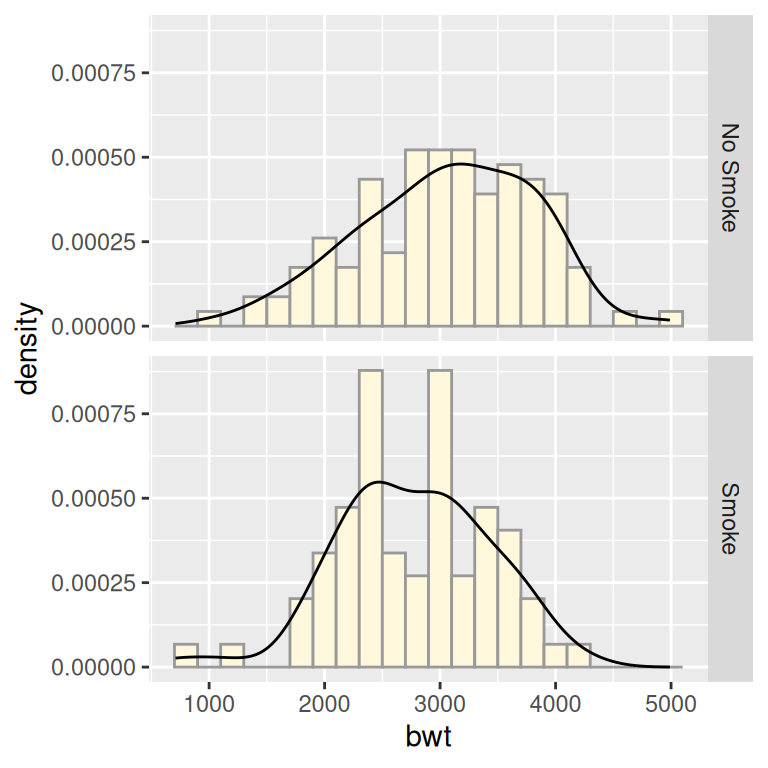Figure 6.14: Density curves overlaid on histograms

## 6.5 Making a Frequency Polygon

### 6.5.1 Problem

You want to make a frequency polygon.

### 6.5.2 Solution

Use geom_`freqpoly()` (Figure 6.15):

``````ggplot(faithful, aes(x=waiting)) +
geom_freqpoly()``````

### 6.5.3 Discussion

A frequency polygon appears similar to a kernel density estimate curve, but it shows the same information as a histogram. That is, like a histogram, it shows what is in the data, whereas a kernel density estimate is just that – an estimate – and requires you to pick some value for the bandwidth.

Like with a histogram, you can control the bin width for the frequency polygon (Figure 6.15, right):

``````ggplot(faithful, aes(x = waiting)) +
geom_freqpoly(binwidth = 4)``````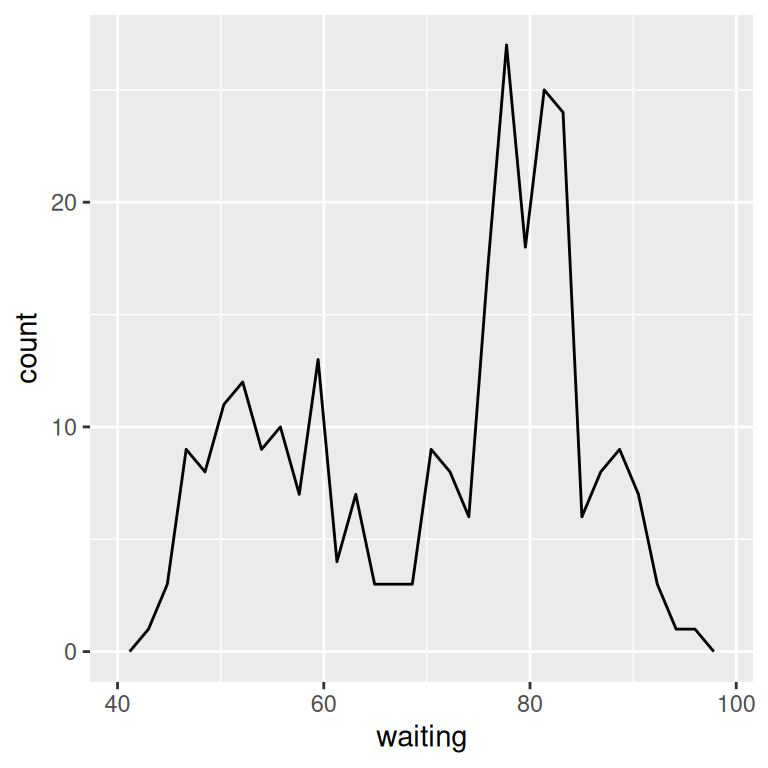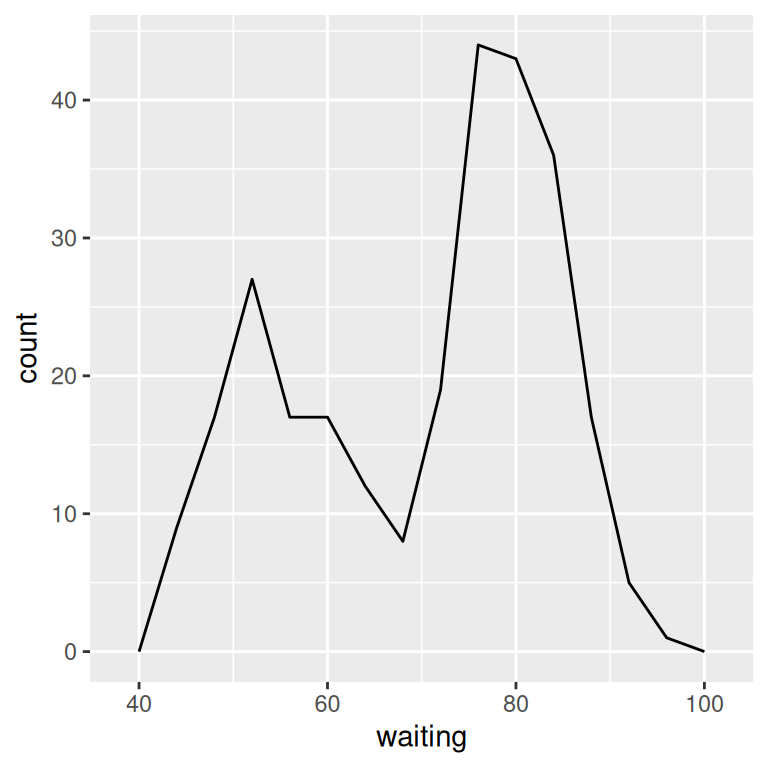Figure 6.15: A frequency polygon (left); With wider bins (right)

Or, instead of setting the width of each bin directly, you can divide the x range into a particular number of bins:

``````# Divide the x-axis range into 15 bins
binsize <- diff(range(faithful\$waiting))/15

ggplot(faithful, aes(x = waiting)) +
geom_freqpoly(binwidth = binsize)``````

Histograms display the same information, but with bars instead of lines. See Recipe 6.1.

## 6.6 Making a Basic Box Plot

### 6.6.1 Problem

You want to make a box (or box-and-whiskers) plot.

### 6.6.2 Solution

Use `geom_boxplot()`, mapping a continuous variable to y and a discrete variable to x (Figure 6.16):

``````library(MASS) # Load MASS for the birthwt data set

# Use factor() to convert a numeric variable into a discrete variable
ggplot(birthwt, aes(x = factor(race), y = bwt)) +
geom_boxplot()``````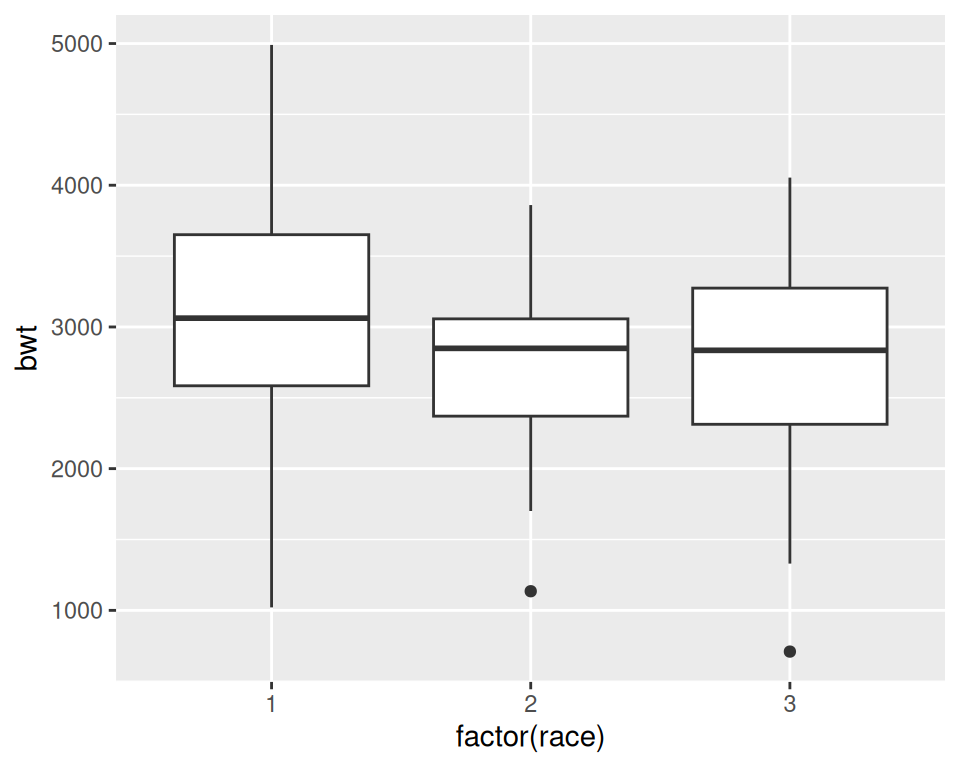Figure 6.16: A box plot

### 6.6.3 Discussion

For this example, we used the `birthwt` data set from the `MASS` package. This data set contains data about birth weights (`bwt`) and a number of risk factors for low birth weight:

``````birthwt
#>    low age lwt race smoke ptl ht ui ftv  bwt
#> 85   0  19 182    2     0   0  0  1   0 2523
#> 86   0  33 155    3     0   0  0  0   3 2551
#> 87   0  20 105    1     1   0  0  0   1 2557
#>  ...<183 more rows>...
#> 82   1  23  94    3     1   0  0  0   0 2495
#> 83   1  17 142    2     0   0  1  0   0 2495
#> 84   1  21 130    1     1   0  1  0   3 2495``````

In Figure 6.16 we have visualized the distributions of `bwt` by each `race` group. Because `race` is stored as a numeric vector with the values of 1, 2, or 3, ggplot doesn’t know how to use this numeric version of `race` as a grouping variable. To make this work, we can modify the data frame by converting `race` to a factor, or by telling ggplot to treat `race` as a factor by using `factor(race)` inside of the `aes()` statement. In the preceding example, we used `factor(race)`.

A box plot consists of a box and “whiskers.” The box goes from the 25th percentile to the 75th percentile of the data, also known as the inter-quartile range (IQR). There’s a line indicating the median, or the 50th percentile of the data. The whiskers start from the edge of the box and extend to the furthest data point that is within 1.5 times the IQR. Any data points that are past the ends of the whiskers are considered outliers and displayed with dots. Figure 6.17 shows the relationship between a histogram, a density curve, and a box plot, using a skewed data set.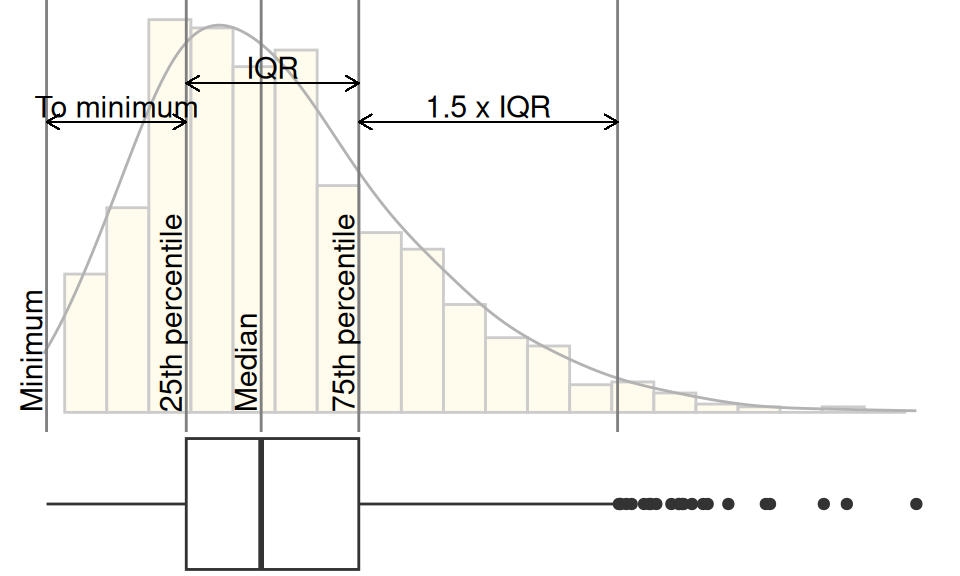Figure 6.17: Box plot compared to histogram and density curve

To change the width of the boxes, you can set width (Figure 6.18, left):

``````ggplot(birthwt, aes(x = factor(race), y = bwt)) +
geom_boxplot(width = .5)``````

If there are many outliers and there is overplotting, you can change the size and shape of the outlier points with `outlier.size` and `outlier.shape`. The default size is 2 and the default shape is 16. This will use smaller points, and hollow circles (Figure 6.18, right):

``````ggplot(birthwt, aes(x = factor(race), y = bwt)) +
geom_boxplot(outlier.size = 1.5, outlier.shape = 21)``````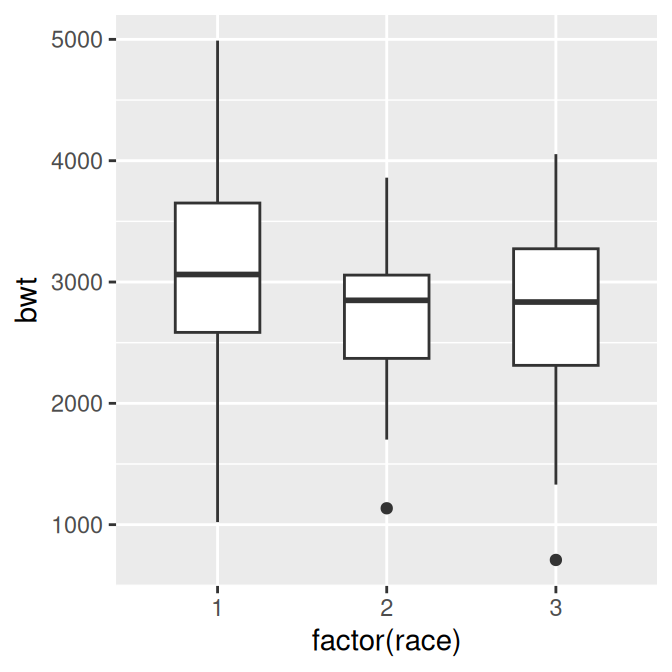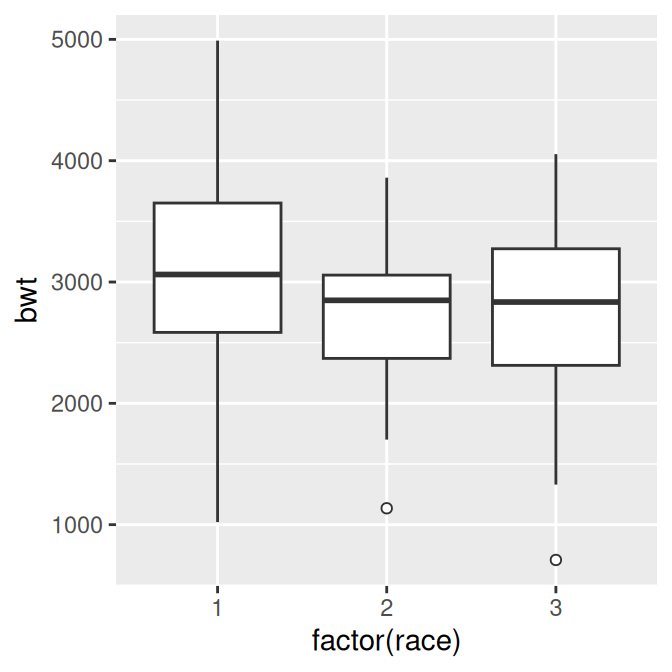Figure 6.18: Box plot with narrower boxes (left); With smaller, hollow outlier points (right)

To make a box plot of just a single group, we have to provide some arbitrary value for x; otherwise, ggplot won’t know what x coordinate to use for the box plot. In this case, we’ll set it to 1 and remove the x-axis tick markers and label (Figure 6.19):

``````ggplot(birthwt, aes(x = 1, y = bwt)) +
geom_boxplot() +
scale_x_continuous(breaks = NULL) +
theme(axis.title.x = element_blank())``````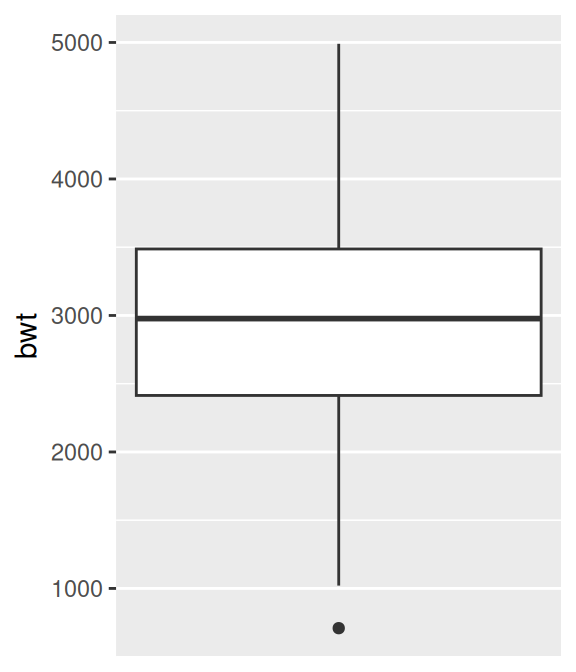Figure 6.19: Box plot of a single group

Note

The calculation of quantiles works slightly differently from the `boxplot()` function in base R. This can sometimes be noticeable for small sample sizes. See `?geom_boxplot` for detailed information about how the calculations differ.

## 6.7 Adding Notches to a Box Plot

### 6.7.1 Problem

You want to add notches to a box plot to assess whether the medians are different.

### 6.7.2 Solution

Use `geom_boxplot()` and set `notch = TRUE` (Figure 6.20):

``````library(MASS) # Load MASS for the birthwt data set

ggplot(birthwt, aes(x = factor(race), y = bwt)) +
geom_boxplot(notch = TRUE)``````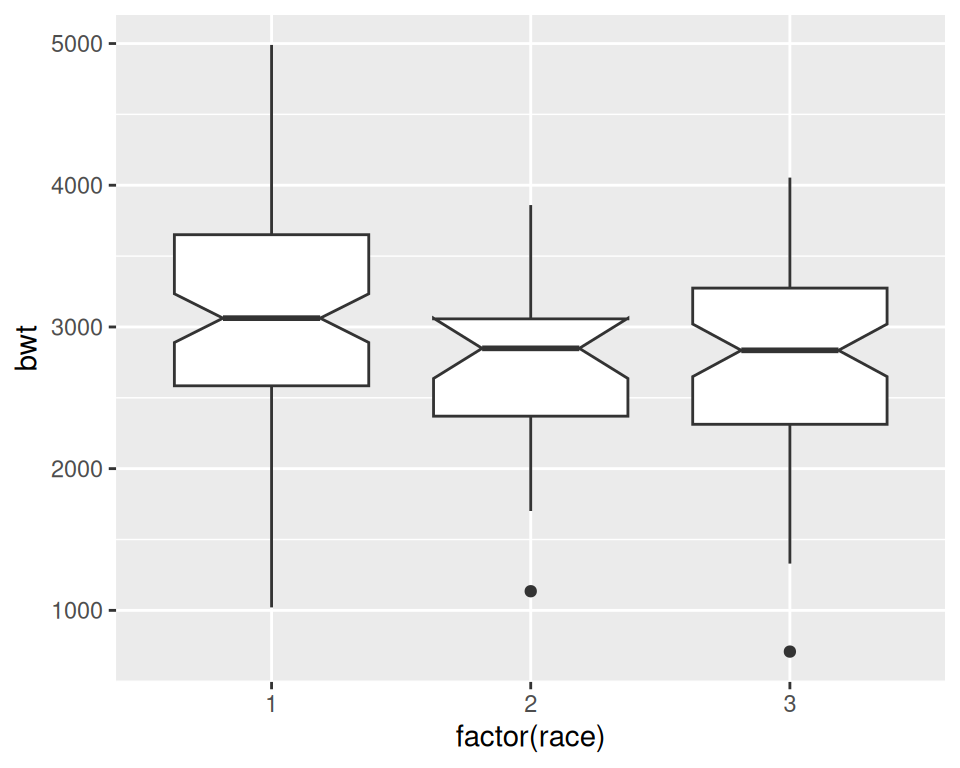Figure 6.20: A notched box plot

### 6.7.3 Discussion

Notches are used in box plots to help visually assess whether the medians of distributions differ. If the notches do not overlap, this is evidence that the medians are different.

With this particular data set, you’ll see the following message:

``Notch went outside hinges. Try setting notch=FALSE.``

This means that the confidence region (the notch) went past the bounds (or hinges) of one of the boxes. In this case, the upper part of the notch in the middle box goes just barely outside the box body, but it’s by such a small amount that you can’t see it in the final output. There’s nothing inherently wrong with a notch going outside the hinges, but it can look strange in more extreme cases.

## 6.8 Adding Means to a Box Plot

### 6.8.1 Problem

You want to add markers for the mean to a box plot.

### 6.8.2 Solution

Use `stat_summary()`. The mean is often shown with a diamond, so we’ll use shape 23 with a white fill. We’ll also make the diamond slightly larger by setting `size = 3` (Figure 6.21):

``````library(MASS) # Load MASS for the birthwt data set

ggplot(birthwt, aes(x = factor(race), y = bwt)) +
geom_boxplot() +
stat_summary(fun.y = "mean", geom = "point", shape = 23, size = 3, fill = "white")``````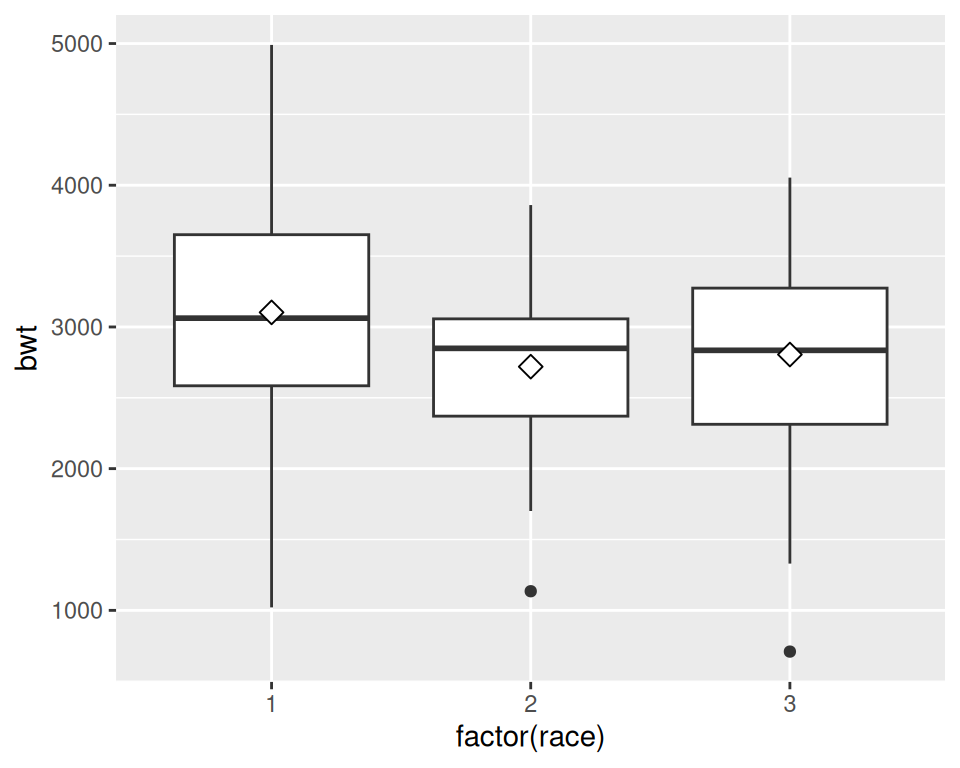Figure 6.21: Mean markers on a box plot

### 6.8.3 Discussion

The horizontal line in the middle of a box plot displays the median, not the mean. For data that is normally distributed, the median and mean will be about the same, but for skewed data these values will differ.

## 6.9 Making a Violin Plot

### 6.9.1 Problem

You want to make a violin plot to compare density estimates of different groups.

### 6.9.2 Solution

Use `geom_violin()` (Figure 6.22):

``````library(gcookbook) # Load gcookbook for the heightweight data set

# Create a base plot using the heightweight data set
hw_p <- ggplot(heightweight, aes(x = sex, y = heightIn))

hw_p +
geom_violin()``````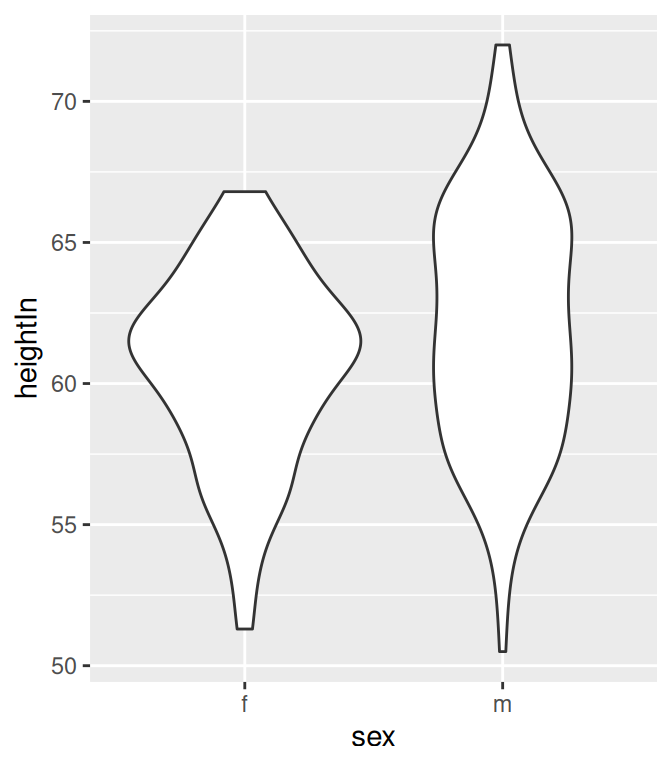Figure 6.22: A violin plot

### 6.9.3 Discussion

Violin plots are a way of comparing multiple data distributions. With ordinary density curves, it is difficult to compare more than just a few distributions because the lines visually interfere with each other. With a violin plot, it’s easier to compare several distributions since they’re placed side by side.

A violin plot is a kernel density estimate, mirrored so that it forms a symmetrical shape. Traditionally, they also have narrow box plots overlaid, with a white dot at the median, as shown in Figure 6.23. Additionally, the box plot outliers are not displayed, which we do by setting `outlier.colour = NA`:

``````hw_p +
geom_violin() +
geom_boxplot(width = .1, fill = "black", outlier.colour = NA) +
stat_summary(fun.y = median, geom = "point", fill = "white", shape = 21, size = 2.5)``````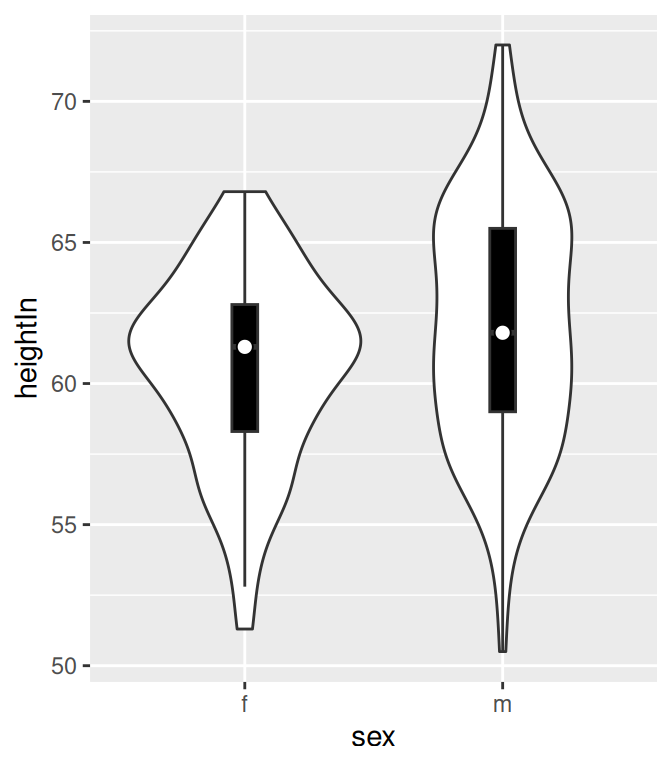Figure 6.23: A violin plot with box plot overlaid on it

In this example we layered the objects from the bottom up, starting with the violin, then the box plot, then the white dot at the median, which is calculated using `stat_summary()`.

The default range goes from the minimum to maximum data values; the flat ends of the violins are at the extremes of the data. It’s possible to keep the tails, by setting `trim = FALSE` (Figure 6.24):

``````hw_p +
geom_violin(trim = FALSE)``````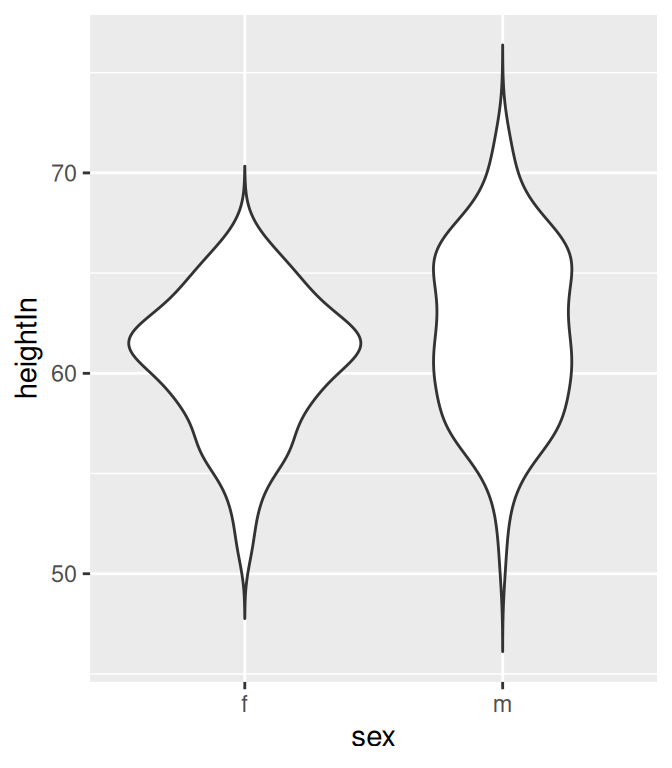Figure 6.24: A violin plot with tails

By default, the violins are scaled so that the total area of each one is the same (if `trim = TRUE`, then it scales what the area would be including the tails). Instead of equal areas, you can use `scale = "count"` to scale the areas proportionally to the number of observations in each group (Figure 6.25). In this example, there are slightly fewer females than males, so the female violin becomes slightly narrower than before:

``````# Scaled area proportional to number of observations
hw_p +
geom_violin(scale = "count")``````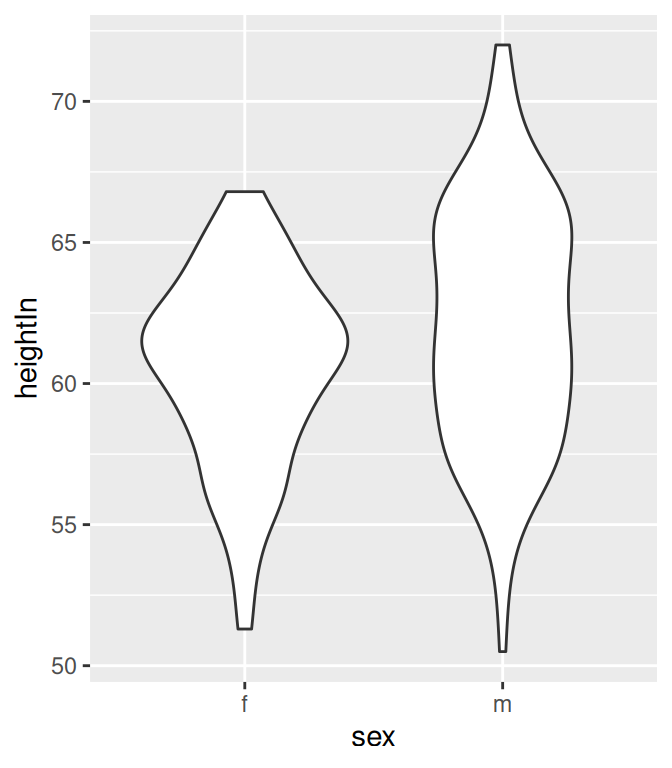Figure 6.25: Violin plot with area proportional to number of observations

To change the amount of smoothing, use the adjust parameter, as described in Recipe 6.3. The default value is 1; use larger values for more smoothing and smaller values for less smoothing (Figure 6.26):

``````# More smoothing
hw_p +

# Less smoothing
hw_p +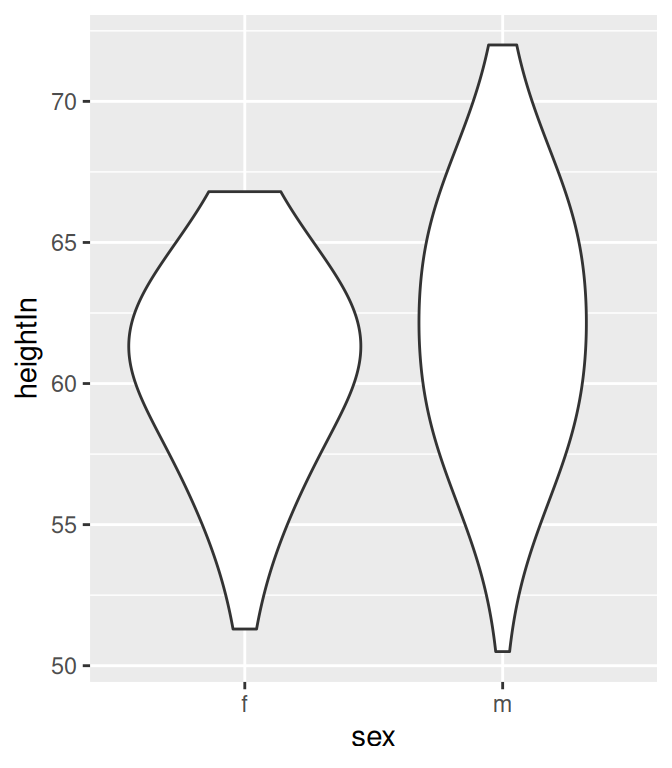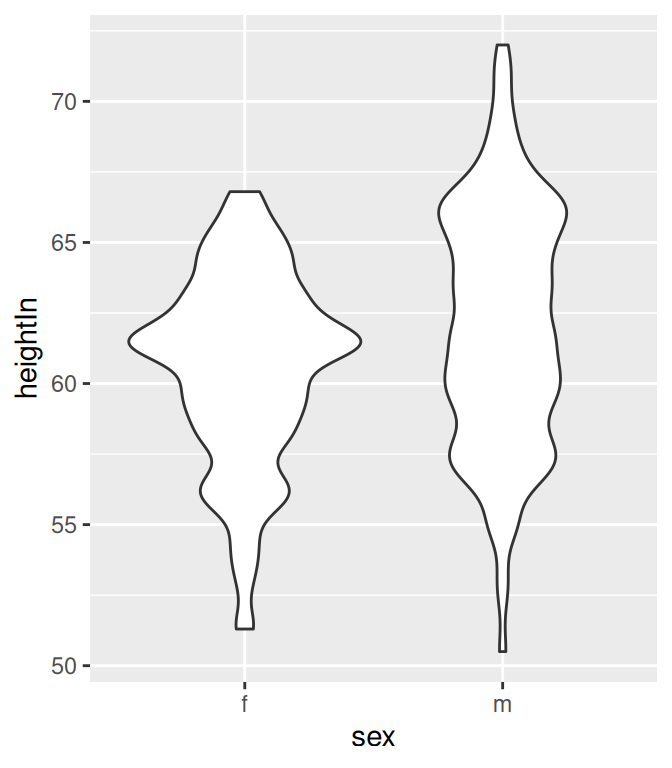Figure 6.26: Violin plot with more smoothing (left); With less smoothing (right)

To create a traditional density curve, see Recipe 6.3.

To use different point shapes, see Recipe 4.5.

## 6.10 Making a Dot Plot

### 6.10.1 Problem

You want to make a Wilkinson dot plot, which shows each data point.

### 6.10.2 Solution

Use `geom_dotplot()`. For this example (Figure 6.27), we’ll use a subset of the `countries` data set:

``````library(gcookbook)  # Load gcookbook for the countries data set
library(dplyr)

# Save a modified data set that only includes 2009 data for countries that
# spent > 2000 USD per capita
c2009 <- countries %>%
filter(Year == 2009 & healthexp > 2000)

# Create a base ggplot object using `c2009`, called `c2009_p` (for c2009 plot)
c2009_p <- ggplot(c2009, aes(x = infmortality))

c2009_p +
geom_dotplot()``````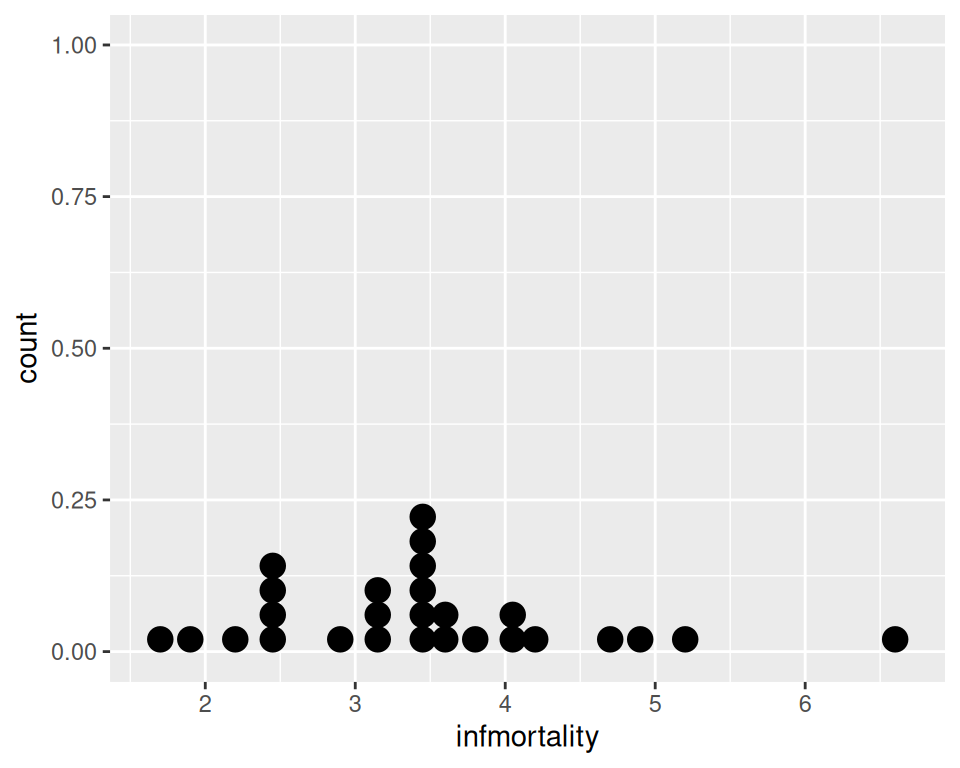Figure 6.27: A dot plot

### 6.10.3 Discussion

This kind of dot plot is sometimes called a Wilkinson dot plot. It’s different from the Cleveland dot plots shown in Recipe 3.10. In these Wilkinson dot plots, the placement of the bins depends on the data, and the width of each dot corresponds to the maximum width of each bin. The maximum bin size defaults to 1/30 of the range of the data, but it can be changed with binwidth.

By default, `geom_dotplot()` bins the data along the x-axis and stacks on the y-axis. The dots are stacked visually, and due to technical limitations of ggplot2, the resulting graph has y-axis tick marks that aren’t meaningful. The y-axis labels can be removed by using `scale_y_continuous()`. In this example, we’ll also use `geom_rug()` to show exactly where each data point is (Figure 6.28):

``````c2009_p +
geom_dotplot(binwidth = .25) +
geom_rug() +
scale_y_continuous(breaks = NULL) +   # Remove tick markers
theme(axis.title.y = element_blank()) # Remove axis label``````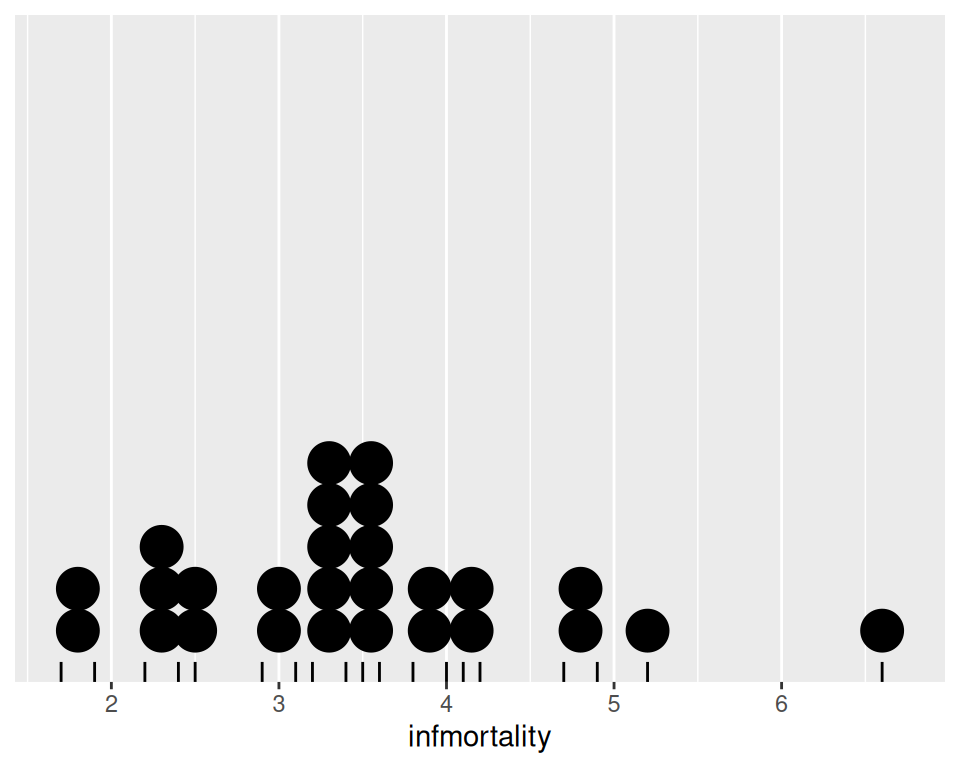Figure 6.28: Dot plot with no y labels, max bin size of .25, and a rug showing each data point

You may notice that the stacks aren’t regularly spaced in the horizontal direction. With the default dotdensity binning algorithm, the position of each stack is centered above the set of data points that it represents. To use bins that are arranged with a fixed, regular spacing, like a histogram, use `method = "histodot"`. In Figure 6.29, you’ll notice that the stacks aren’t centered above the data:

``````c2009_p +
geom_dotplot(method = "histodot", binwidth = .25) +
geom_rug() +
scale_y_continuous(breaks = NULL) +
theme(axis.title.y = element_blank())``````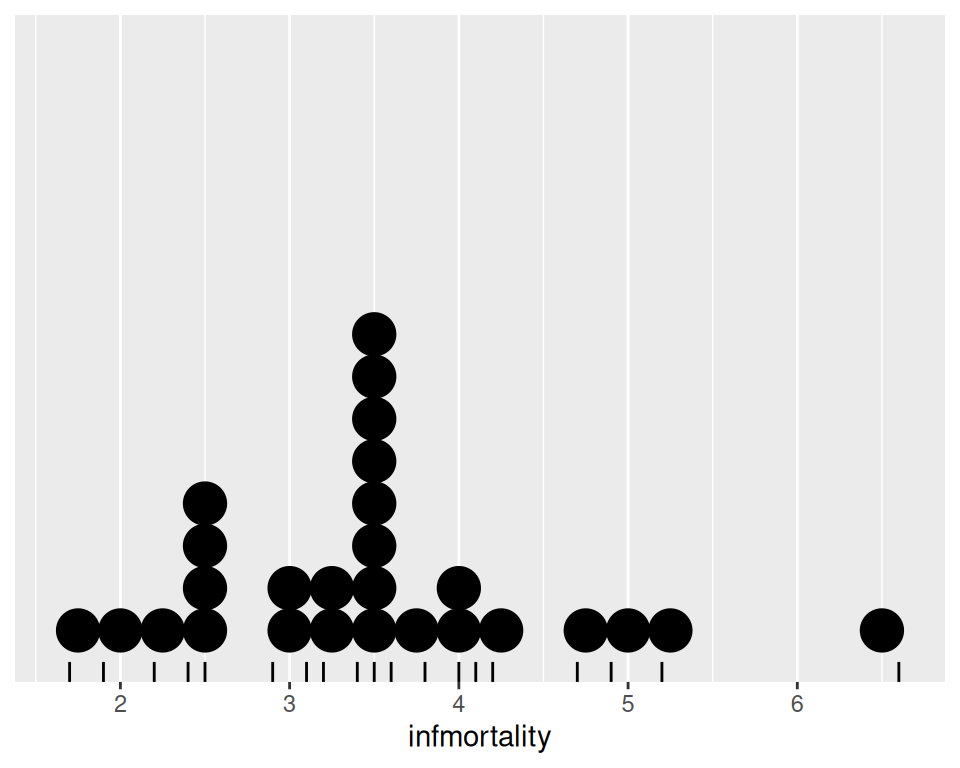Figure 6.29: Dot plot with histodot (fixed-width) binning

The dots can also be stacked centered, or centered in such a way that stacks with even and odd quantities stay aligned. This can by done by setting `stackdir = "center"` or `stackdir = "centerwhole"`, as illustrated in Figure 6.30:

``````c2009_p +
geom_dotplot(binwidth = .25, stackdir = "center") +
scale_y_continuous(breaks = NULL) +
theme(axis.title.y = element_blank())

c2009_p +
geom_dotplot(binwidth = .25, stackdir = "centerwhole") +
scale_y_continuous(breaks = NULL) +
theme(axis.title.y = element_blank())``````Figure 6.30: Dot plot with stackdir = “center” (left); With stackdir = “centerwhole” (right)

Leland Wilkinson, “Dot Plots,” The American Statistician 53 (1999): 276–281, https://www.cs.uic.edu/~wilkinson/Publications/dotplots.pdf.

## 6.11 Making Multiple Dot Plots for Grouped Data

### 6.11.1 Problem

You want to make multiple dot plots from grouped data.

### 6.11.2 Solution

To compare multiple groups, it’s possible to stack the dots along the y-axis, and group them along the x-axis, by setting `binaxis = "y"`. For this example, we’ll use the heightweight data set (Figure 6.31):

``````library(gcookbook) # Load gcookbook for the heightweight data set

ggplot(heightweight, aes(x = sex, y = heightIn)) +
geom_dotplot(binaxis = "y", binwidth = .5, stackdir = "center")``````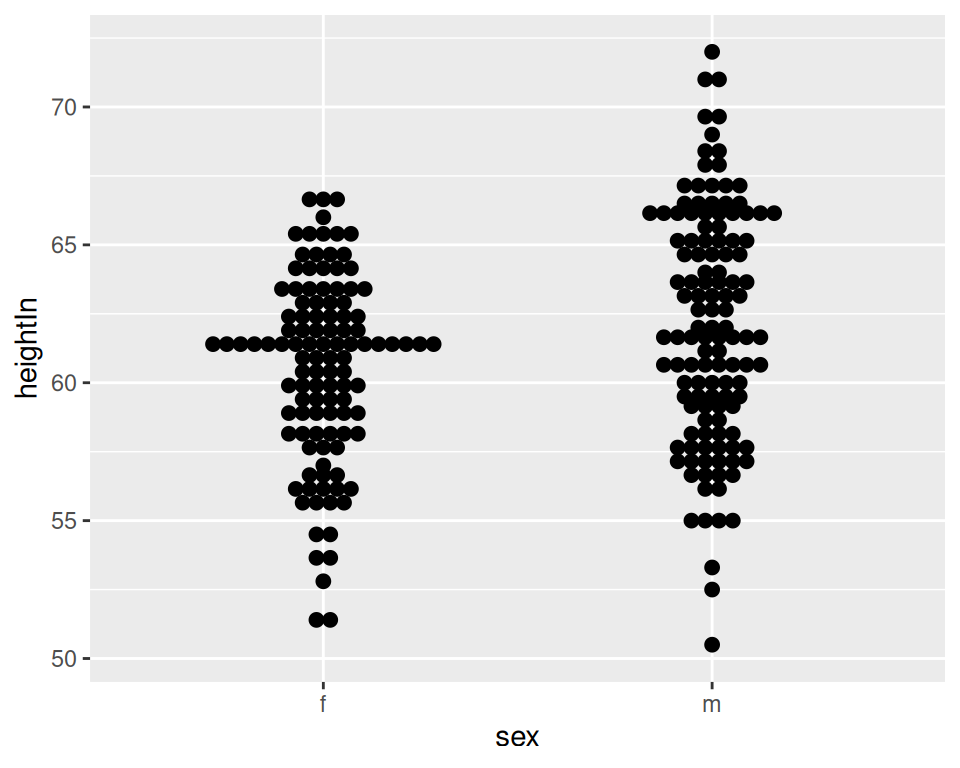Figure 6.31: Dot plot of multiple groups, binning along the y-axis

### 6.11.3 Discussion

Dot plots are sometimes overlaid on box plots. In these cases, it may be helpful to make the dots hollow and have the box plots not show outliers, since the outlier points will appear to be part of the dot plot (Figure 6.32):

``````ggplot(heightweight, aes(x = sex, y = heightIn)) +
geom_boxplot(outlier.colour = NA, width = .4) +
geom_dotplot(binaxis = "y", binwidth = .5, stackdir = "center", fill = NA)``````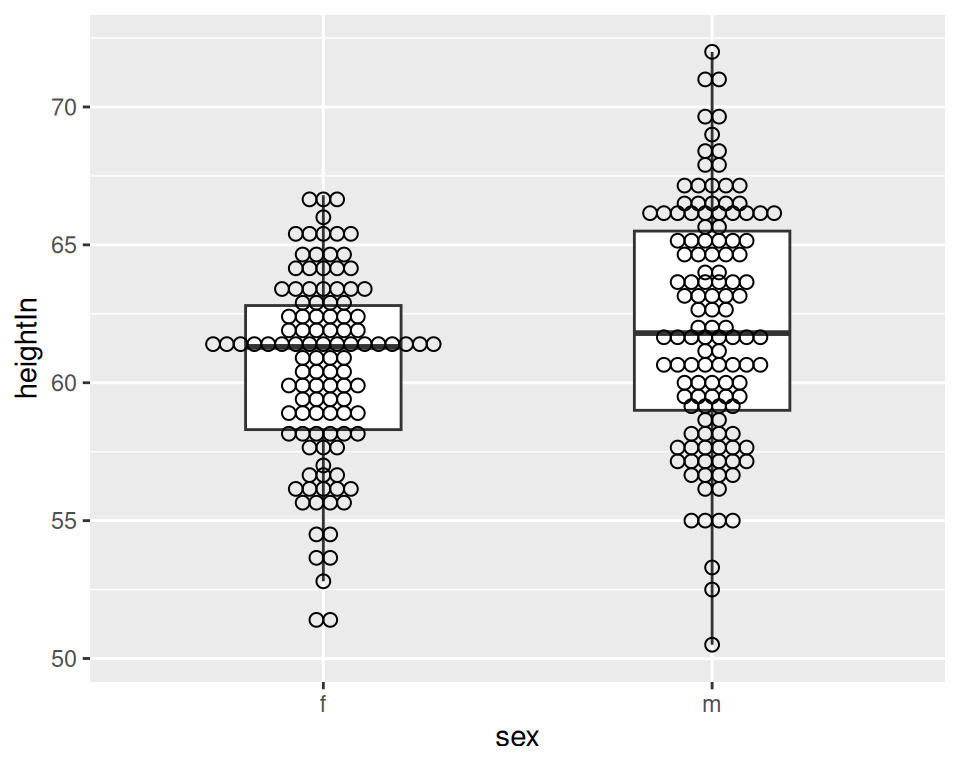Figure 6.32: Dot plot overlaid on box plot

It’s also possible to show the dot plots next to the box plots, as shown in Figure 6.33. This requires using a bit of a hack, by treating the x variable as a numeric variable and then subtracting or adding a small quantity to shift the box plots and dot plots left and right. When the x variable is treated as numeric you must also specify the group, or else the data will be treated as a single group, with just one box plot and dot plot. Finally, since the x-axis is treated as numeric, it will by default show numbers for the x-axis tick labels; they must be modified with `scale_x_continuous()` to show x tick labels as text corresponding to the factor levels:

``````ggplot(heightweight, aes(x = sex, y = heightIn)) +
geom_boxplot(aes(x = as.numeric(sex) + .2, group = sex), width = .25) +
geom_dotplot(
aes(x = as.numeric(sex) - .2, group = sex),
binaxis = "y",
binwidth = .5,
stackdir = "center"
) +
scale_x_continuous(
breaks = 1:nlevels(heightweight\$sex),
labels = levels(heightweight\$sex)
)``````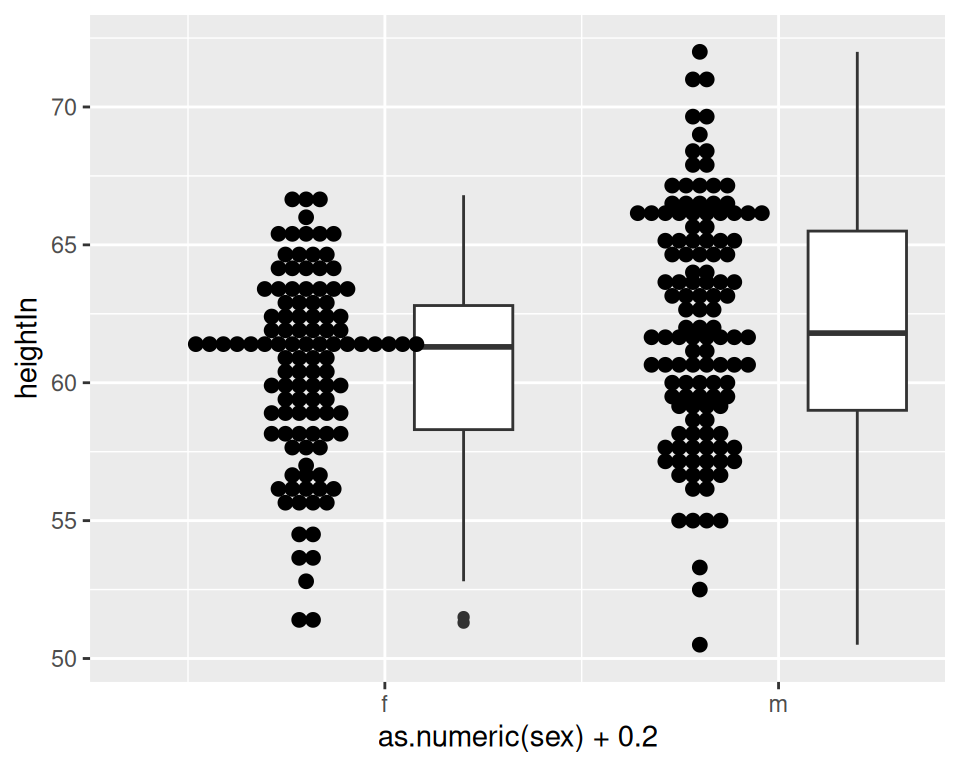Figure 6.33: Dot plot next to box plot

## 6.12 Making a Density Plot of Two-Dimensional Data

### 6.12.1 Problem

You want to plot the density of two-dimensional data.

### 6.12.2 Solution

Use `stat_density2d()`. This makes a 2D kernel density estimate from the data. First we’ll plot the density contour along with the data points (Figure 6.34, left):

``````# Save a base plot object
faithful_p <- ggplot(faithful, aes(x = eruptions, y = waiting))

faithful_p +
geom_point() +
stat_density2d()``````

It’s also possible to map the height of the density curve to the color of the contour lines, by using `..level..` (Figure 6.34, right):

``````# Contour lines, with "height" mapped to color
faithful_p +
stat_density2d(aes(colour = ..level..))``````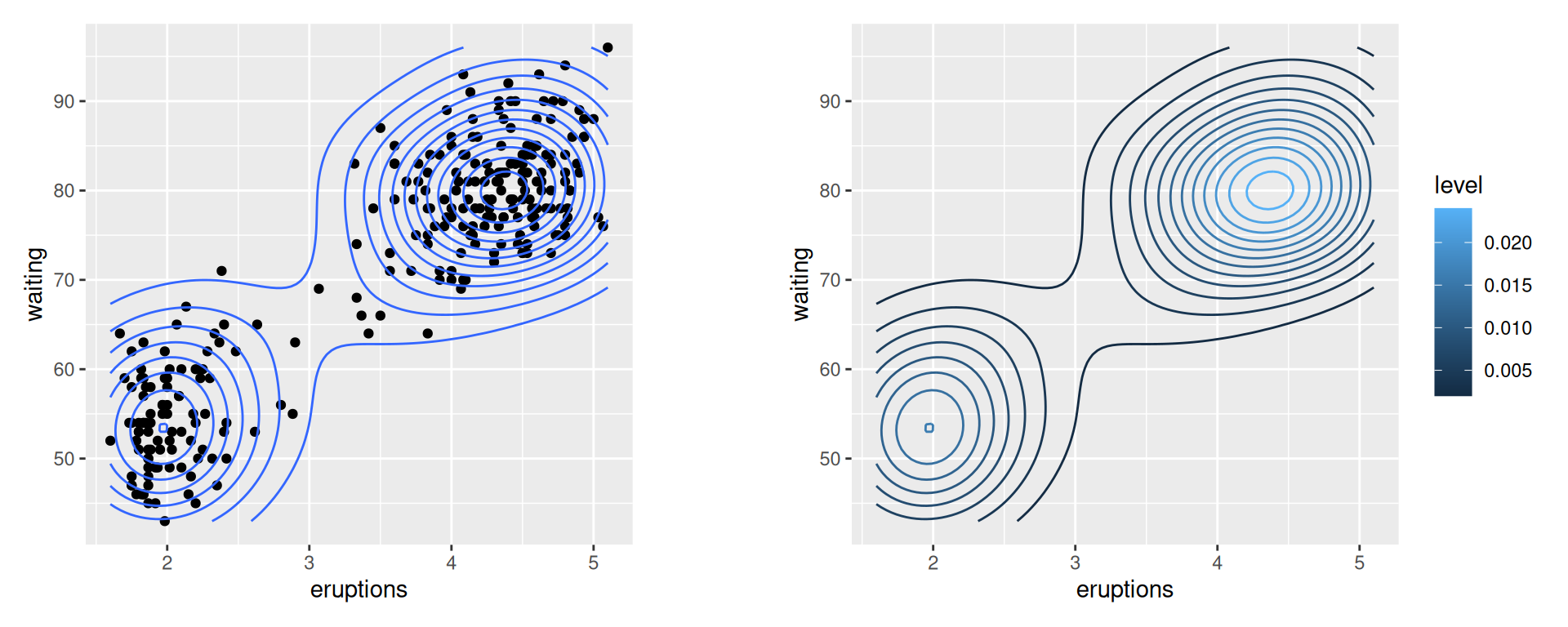Figure 6.34: Points and density contour (left); With ..level.. mapped to color (right)

### 6.12.3 Discussion

The two-dimensional kernel density estimate is analogous to the one-dimensional density estimate generated by `stat_density()`, but of course, it needs to be viewed in a different way. The default is to use contour lines, but it’s also possible to use tiles and to map the density estimate to the fill color, or to the transparency of the tiles, as shown in Figure 6.35:

``````# Map density estimate to fill color
faithful_p +
stat_density2d(aes(fill = ..density..), geom = "raster", contour = FALSE)

# With points, and map density estimate to alpha
faithful_p +
geom_point() +
stat_density2d(aes(alpha = ..density..), geom = "tile", contour = FALSE)``````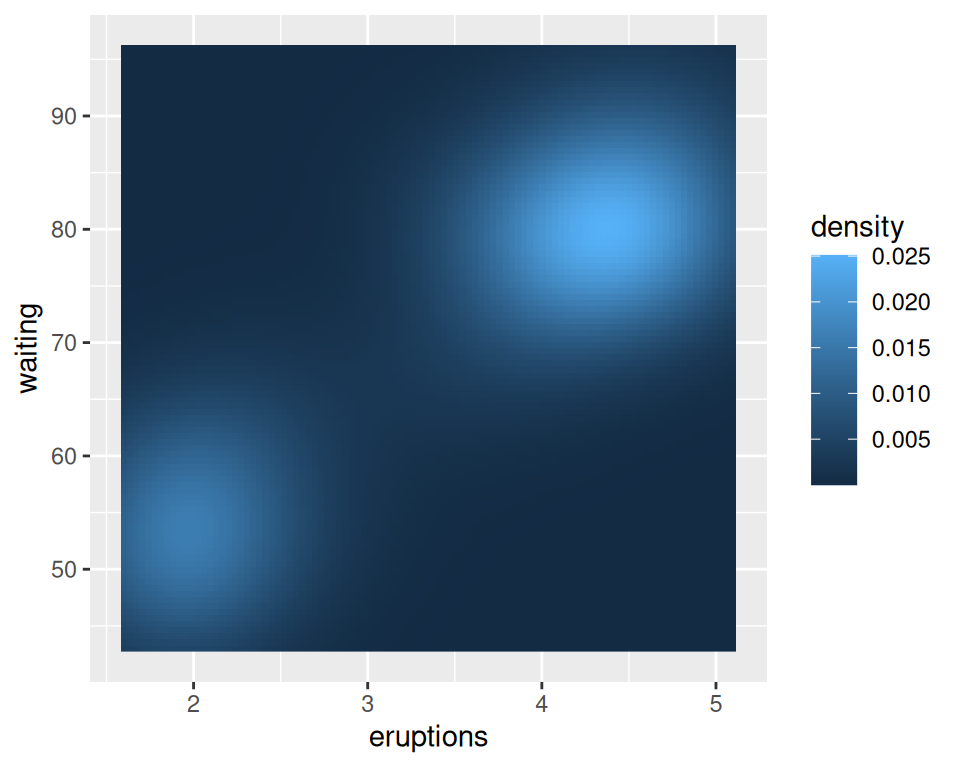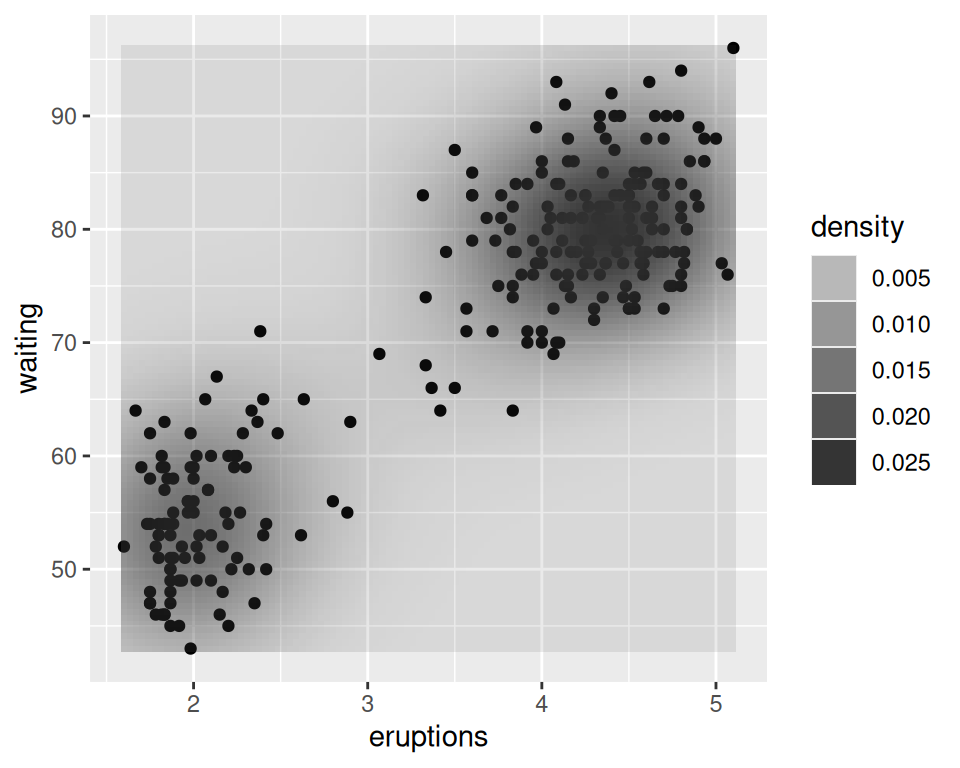Figure 6.35: With `..density..` mapped to fill (left); With points, and ..density.. mapped to alpha (right)

Note

We used `geom = "raster"` in the first of the preceding examples and `geom = "tile"` in the second. The main difference is that the raster geom renders more efficiently than the tile geom. In theory they should appear the same, but in practice they often do not. If you are writing to a PDF file, the appearance depends on the PDF viewer. On some viewers, when tile is used there may be faint lines between the tiles, and when raster is used the edges of the tiles may appear blurry (although it doesn’t matter in this particular case).

As with the one-dimensional density estimate, you can control the bandwidth of the estimate. To do this, pass a vector for the x and y bandwidths to `h`. This argument gets passed on to the function that actually generates the density estimate, `kde2d()`. In this example (Figure 6.36), we’ll use a smaller bandwidth in the x and y directions, so that the density estimate is more closely fitted (perhaps overfitted) to the data:

``````faithful_p +
stat_density2d(
aes(fill = ..density..),
geom = "raster",
contour = FALSE,
h = c(.5, 5)
)``````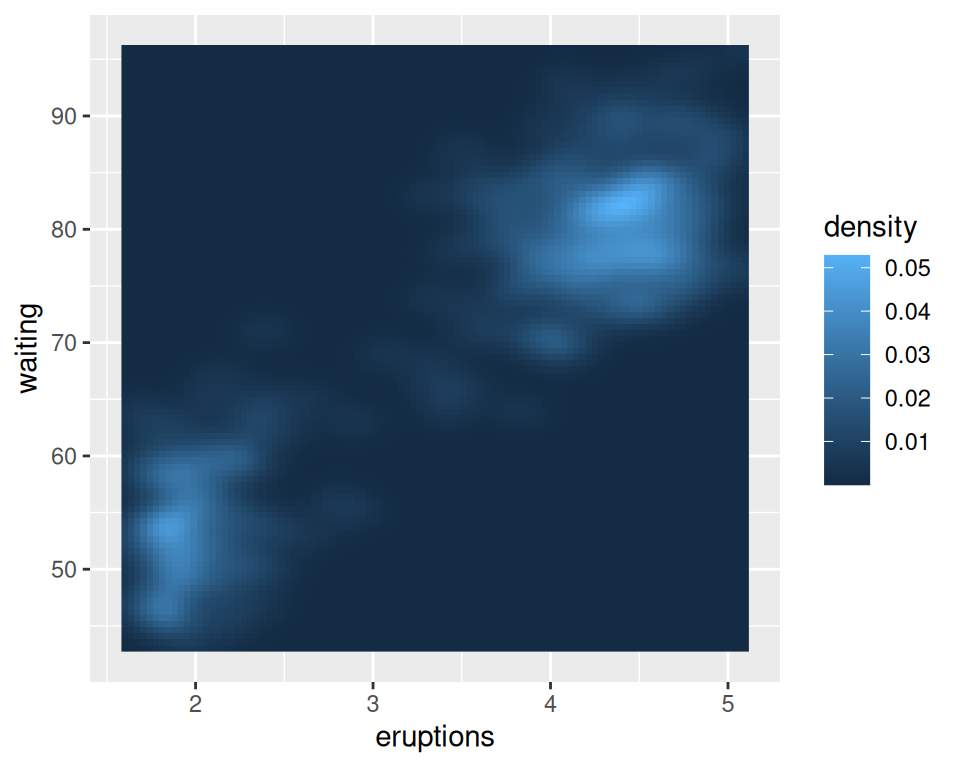Figure 6.36: Density plot with a smaller bandwidth in the x and y directions

The relationship between `stat_density2d()` and `stat_bin2d()` is the same as the relationship between their one-dimensional counterparts, the density curve and the histogram. The density curve is an estimate of the distribution under certain assumptions, while the binned visualization represents the observed data directly. See Recipe 5.5 for more about binning data.
`stat_density2d()` passes options to `kde2d()`; see `?kde2d` for information on the available options.